Select Page

# R Graphics

## The Graphic Environment

R comes with a wide variety of graphical functions. The R default graphics package provide standard R graphics. Additional libraries such as lattice and ggplot provide specialized and often very attractive graphics. This chapter is mainly about classical R graphics. Introductory examples of lattice and ggplot2 graphics will be provided at the end of this chapter.

The graphical functions in the base R system, can be divided into two groups:

• High level plot functions. These functions produce “complete” graphics and will erase existing plots if not specified otherwise.
• Low level plot functions. These functions are used to add graphical objects like lines, points and texts to existing plots.

Generally, high level graphic functions are named according to the corresponding graphics. Simple examples are: barplot(), boxplot(), pie().

A special case is the plot() function. This function is a generic function and perform differently according to its arguments or, more precisely, according to the class of the objects passed as arguments.

As a results: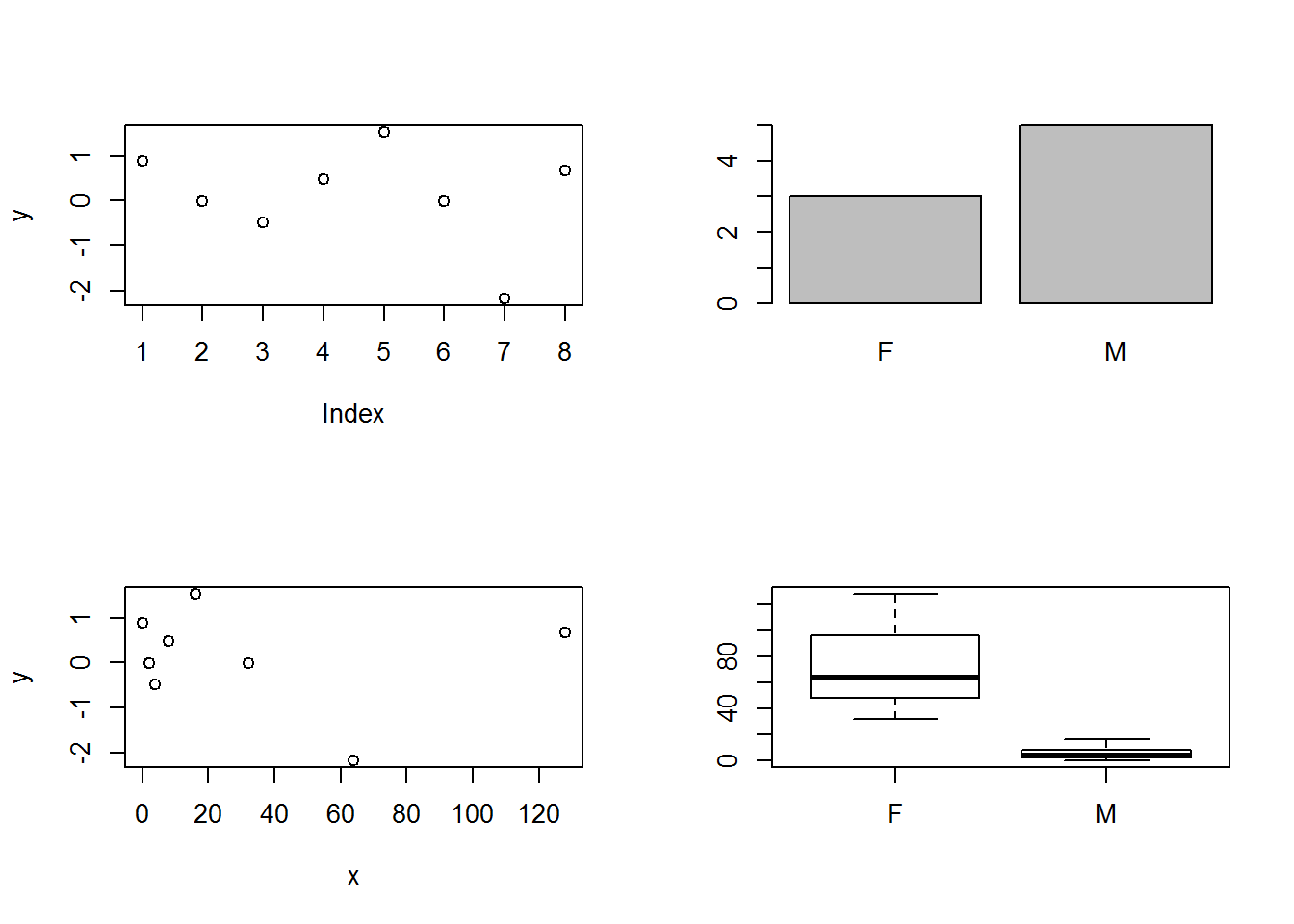## Scatterplot

The scatter plot (or scattergraph) is the main tool for the study of bivariate numerical distributions. $$(x_1, y_1), \dots, (x_n, y_n)$$ indicate the sets of data obtained from the X, Y numeric variables. The scatter plot is a graph where the points $$P_1 = (x_1, y_1), \dots, P_n = (x_n, y_n)$$ are defined in a Cartesian coordinate system. The features of the point cloud, such as location, internal cohesion, direction, and presence of isolated points, enable the deduction of the distribution statistical characteristics (position, dispersion, correlation, anomalous data).

Generally, the plot() function is called to produce simple scatter plot: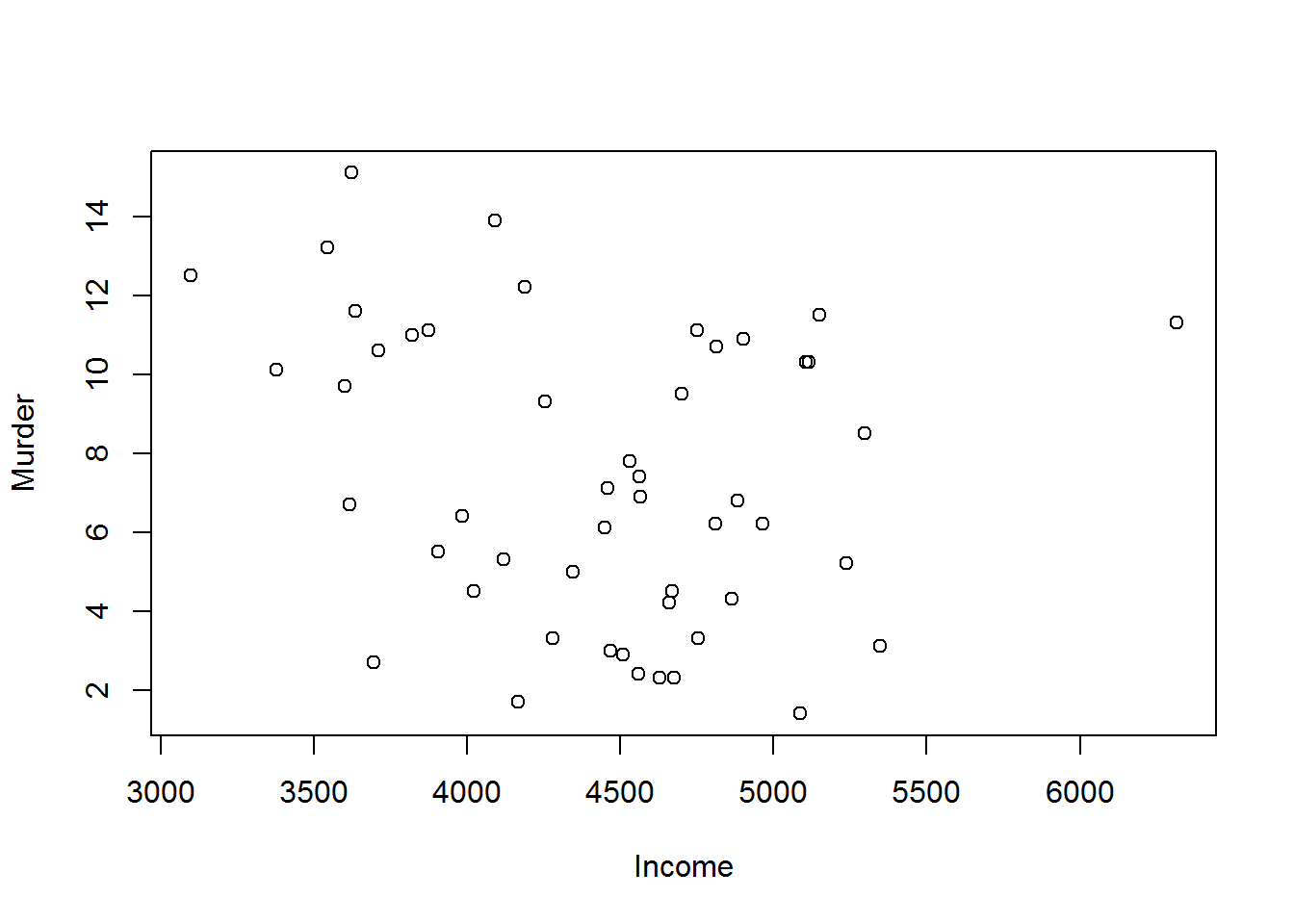An alternative and more elegant way of calling the plot() function consist in specifying the x and y arguments by mean of a formula. Note that this method allows the data argument to be specified as an argument of the plot function.Some models differing from the basic plot are shown below.

• Addition of further elements, such as the centroid (the point whose coordinates are the arithmetic mean of X and Y, and the barycentre of the distribution), the least-squares line, particular concentration ellipses for the bivariate Gaussian distribution.

• Subordination: creation of a scatter plot of a set of variables for each level of the third subordinate variable.

• P-variate numerical distributions, $$p > 2$$: creation of a scatter plot matrix, a pxp square matrix, where the generic cell $$(i, j)$$ outside the main diagonal contains the scatter plot of the $$i$$ and $$j$$ variables, whereas the diagonal cells contain box-and-whiskers plots or histograms.

The scatter plot can be created with the plot() function (scatter plot for a set of variables), but also with pairs() (dispersion matrix) and coplot() (scatter plots of a set of variables for specified levels of a third alphanumeric or numeric variable). Moreover, the locator() and identify() functions enable the interactive use of the plot by adding further elements in the positions indicated by the mouse (locator) and underlying the index or the label of the point closest to the mouse pointer (identify). This function will not be discussed in this document.

### Type

When calling the plot() function, the argument type is set to its default: type = "p". As a results graphics coordinates are represented by points (empty circles). Different graphical representations are given by: "l" for lines, "o" for overplotted points and lines, "b" for both points and lines, "c" for the lines parte alone of "b", "s" and "S" for stair steps and "h" for histogram-like vertical lines. type = "n" is particularly important. In this case an empty plot with axes is created. The plot can be later customized in an extremely sophisticated way using more advanced graphic functions.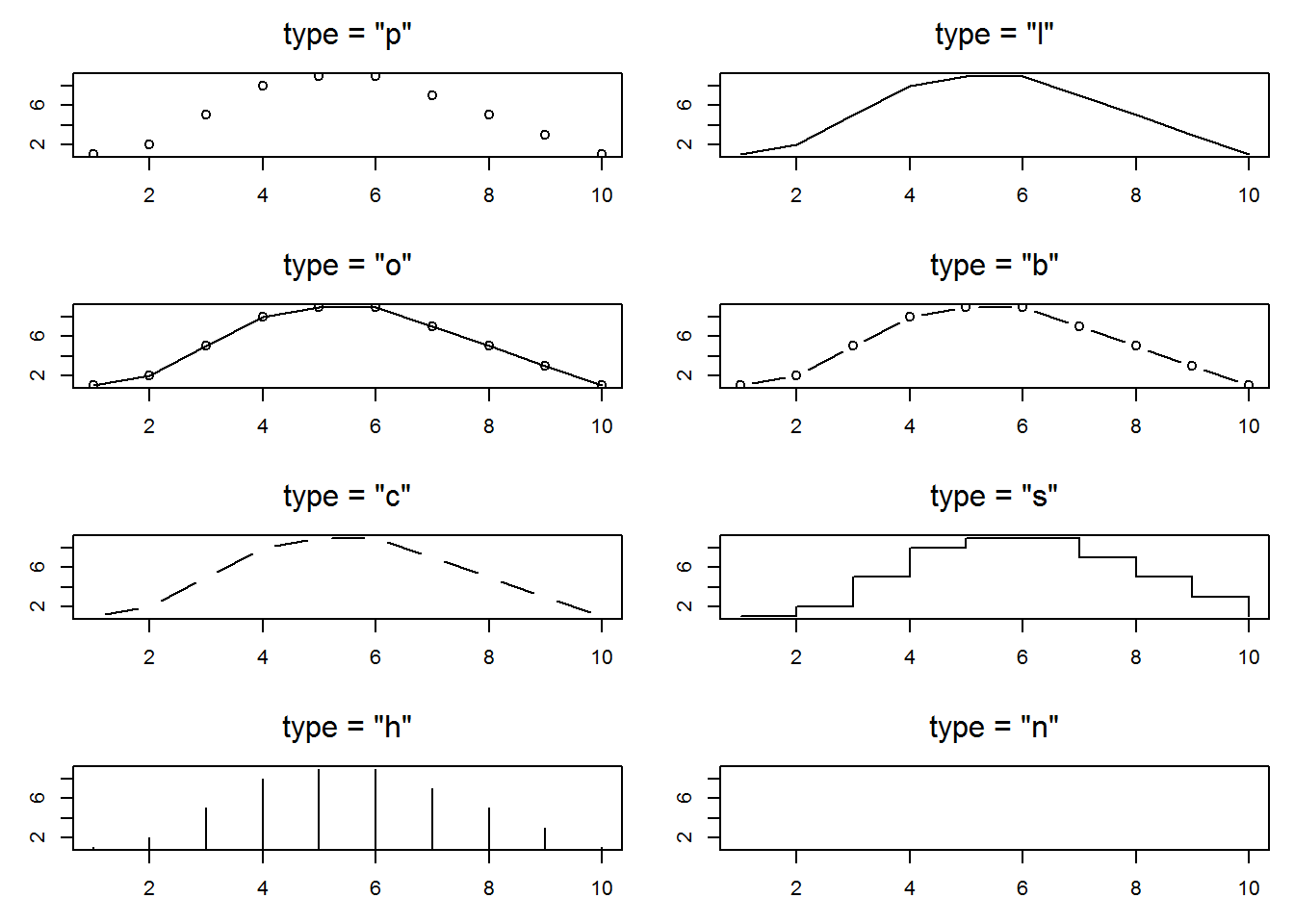### Symbols

During the creation of the plot, the shape, the dimensions and the colour of the symbols can be customized with the pch, cex and col parameters respectively. An example of the implementation of these parameters inside plot() and the output graph are shown below.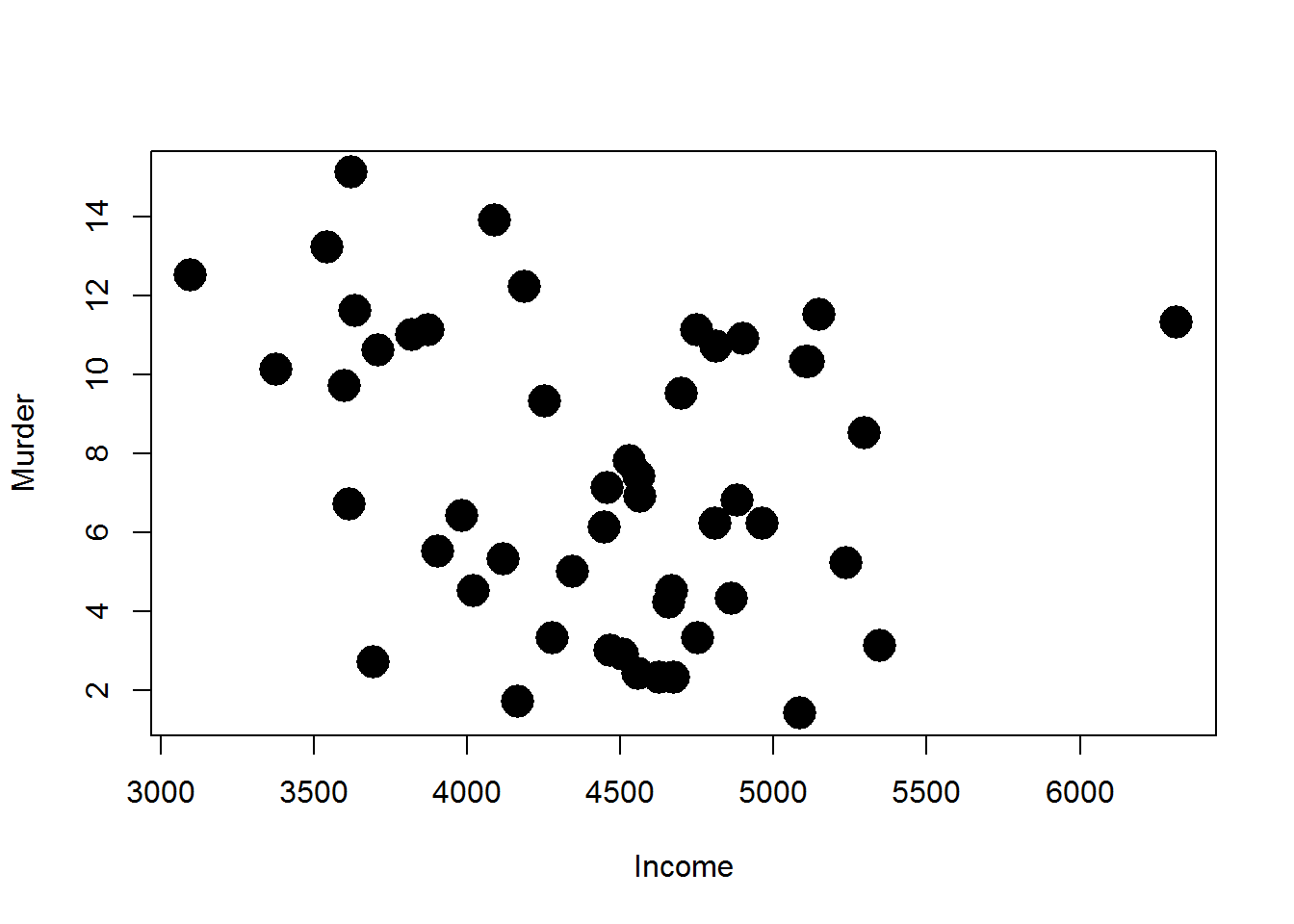There are 25 symbols in R. Figure below shows these symbols and the reference values to be associated with the pch parameter.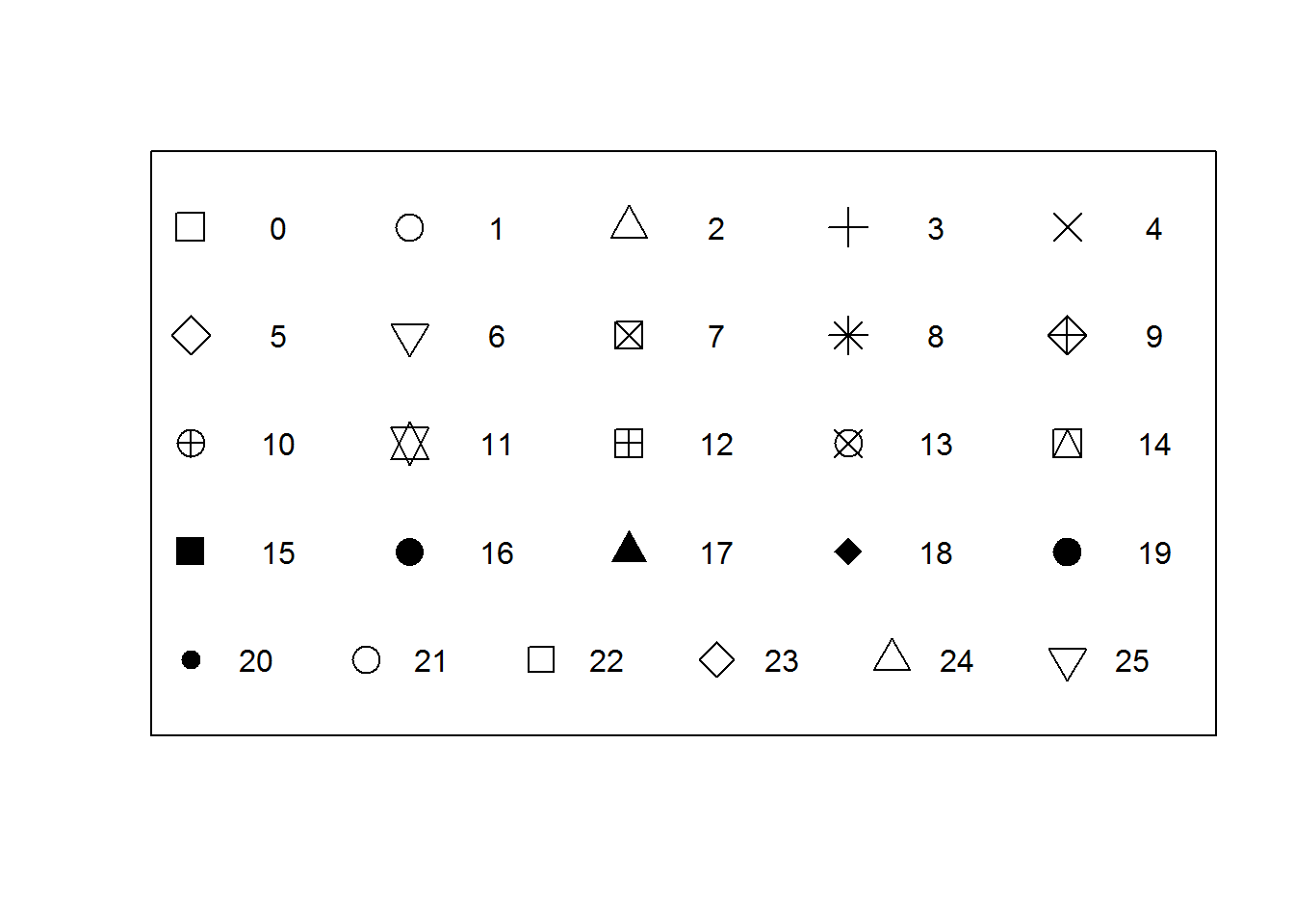If you want to use a symbol which is not one of the standard 25, you can write it explicitly in the pch parameter. It needs to have only one character.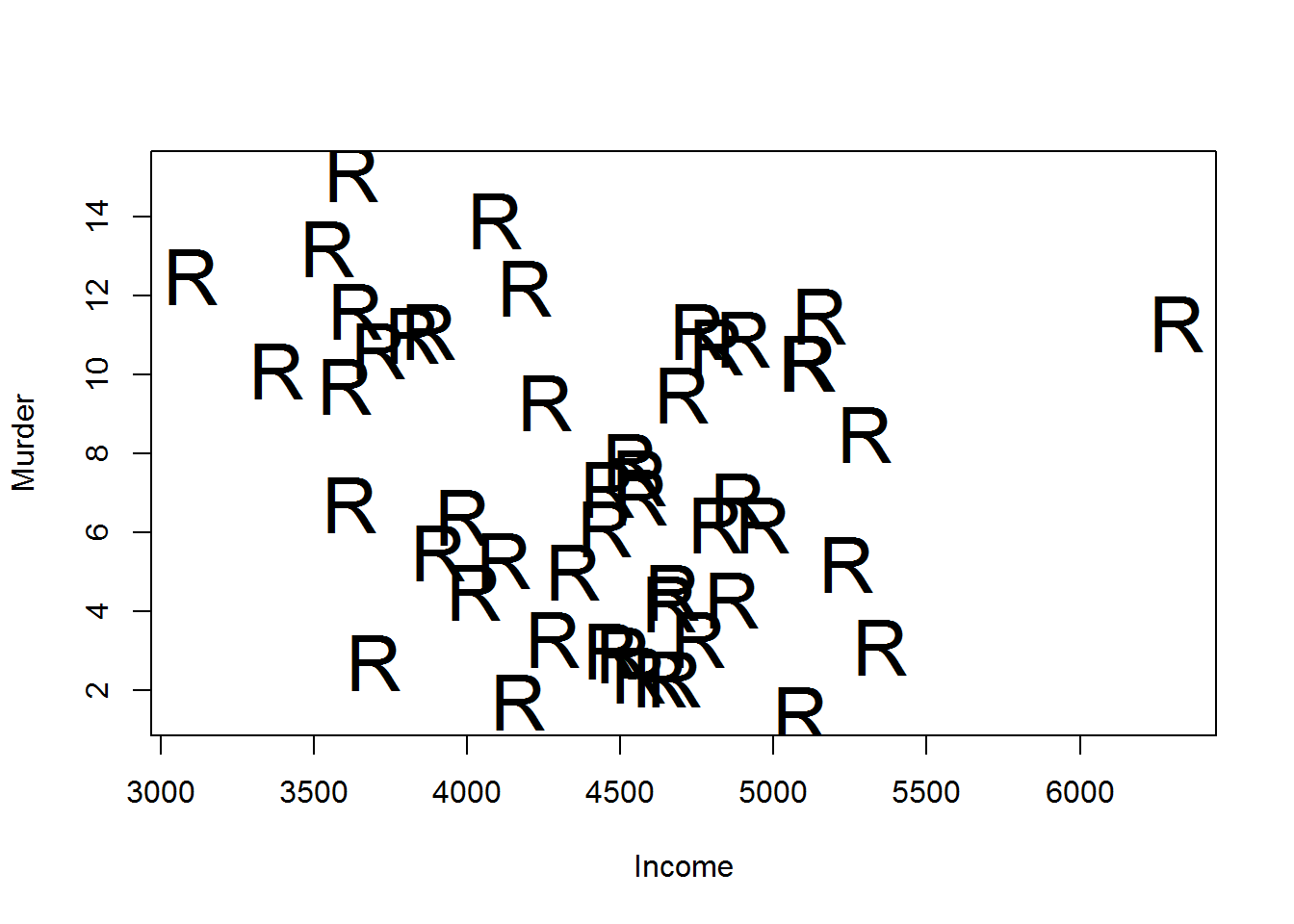The cex parameter increases the dimension of the symbols as much as the parameter value.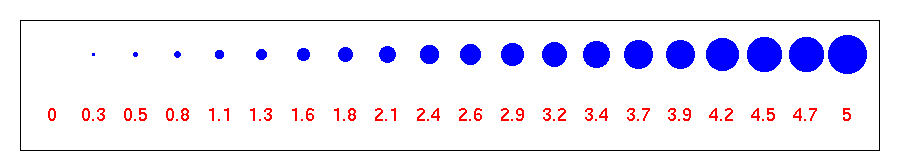### Colours

In R the col parameter manages the colours of the symbols inside the plots. col can be defined in different ways. Some of these methods are as follows:

1. Specification of a number comprised between 1 and 8. In the graph above it is clear that these eight colours are repeated whenever there are multiples of eight.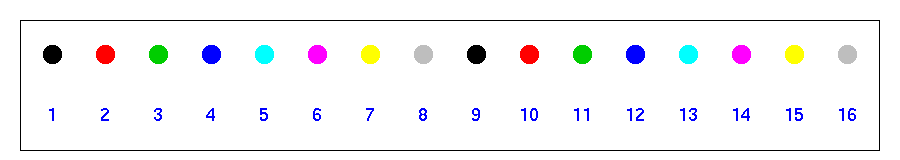2. Specification of the name of the colour in English: red, blue, etc. There are 657 colours which can be defined in this way in R. For a complete list of available colours digit the colors() function, without arguments.
3. Use of the default colour sequence. These sequences are available thanks to some functions in which the input parameter specifies the number of colours to be extracted from the colour space. The above-mentioned functions are rainbow(), heat.colors(), terrain.colors(), topo.colors() and cm.colors().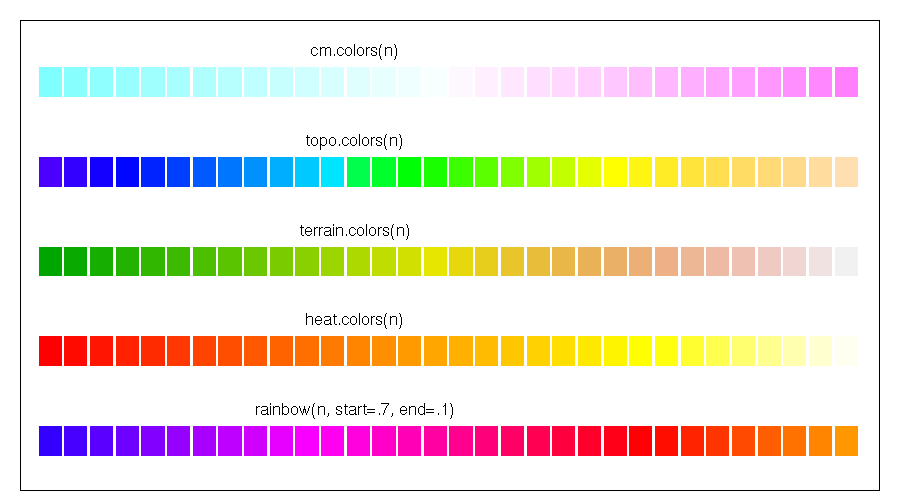4. Specification of the colour in the hexadecimal format: #000000, #ffffff ecc.

### Titles

The main parameter of the plot() function enables the definition of the main title of the plot. This title will be displayed in the top centre of the plot. The sub parameter creates a subtitle which is displayed in the bottom centre of the plot. A title on two or more rows can be defined by inserting the special character “\n” in the title string. Finally, the xlab and ylab parameters change the titles of the x and y axes respectively.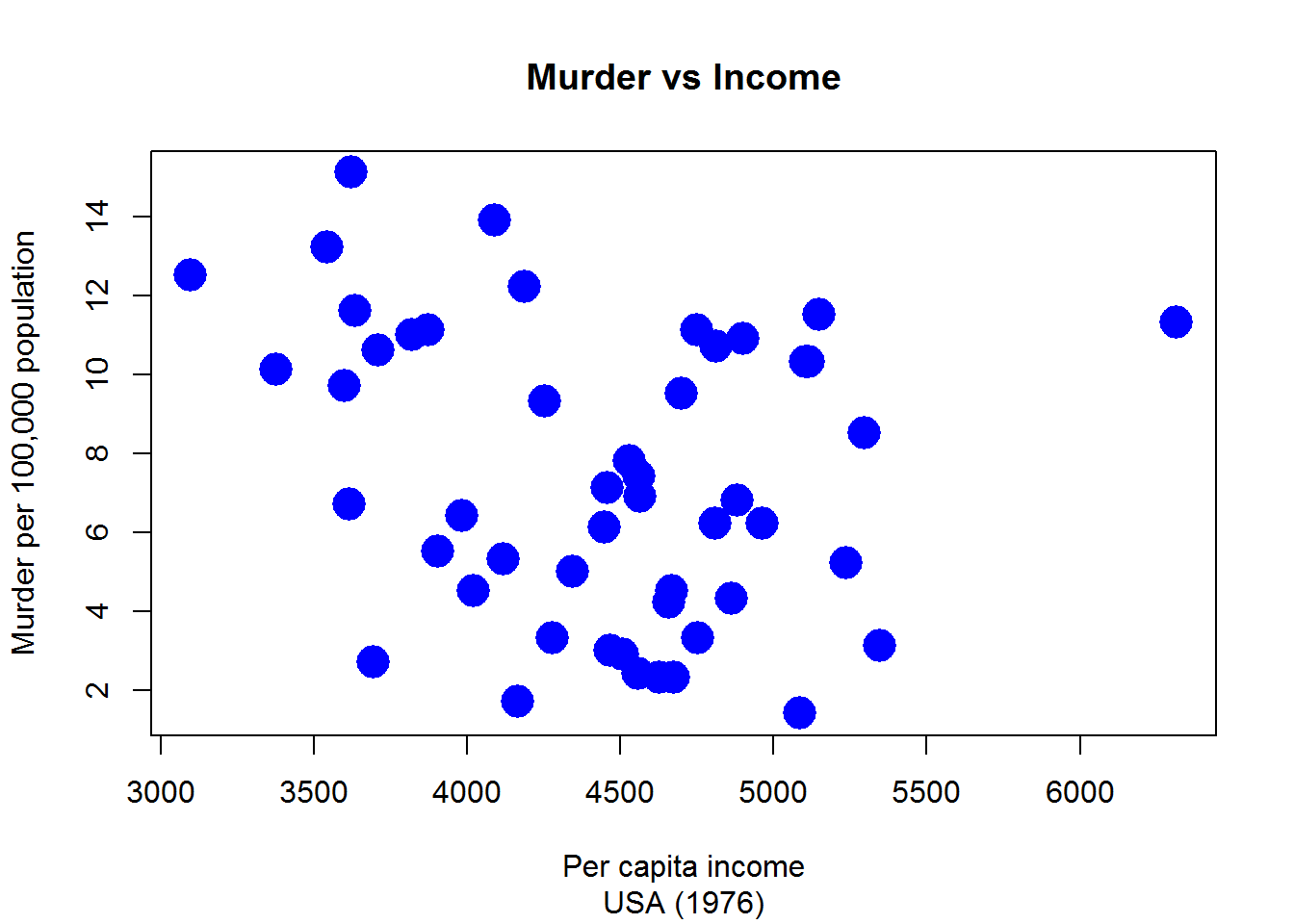### Axes

The xlim parameter sets a range for the x-axis. The ylim parameter sets a range for the y-axis.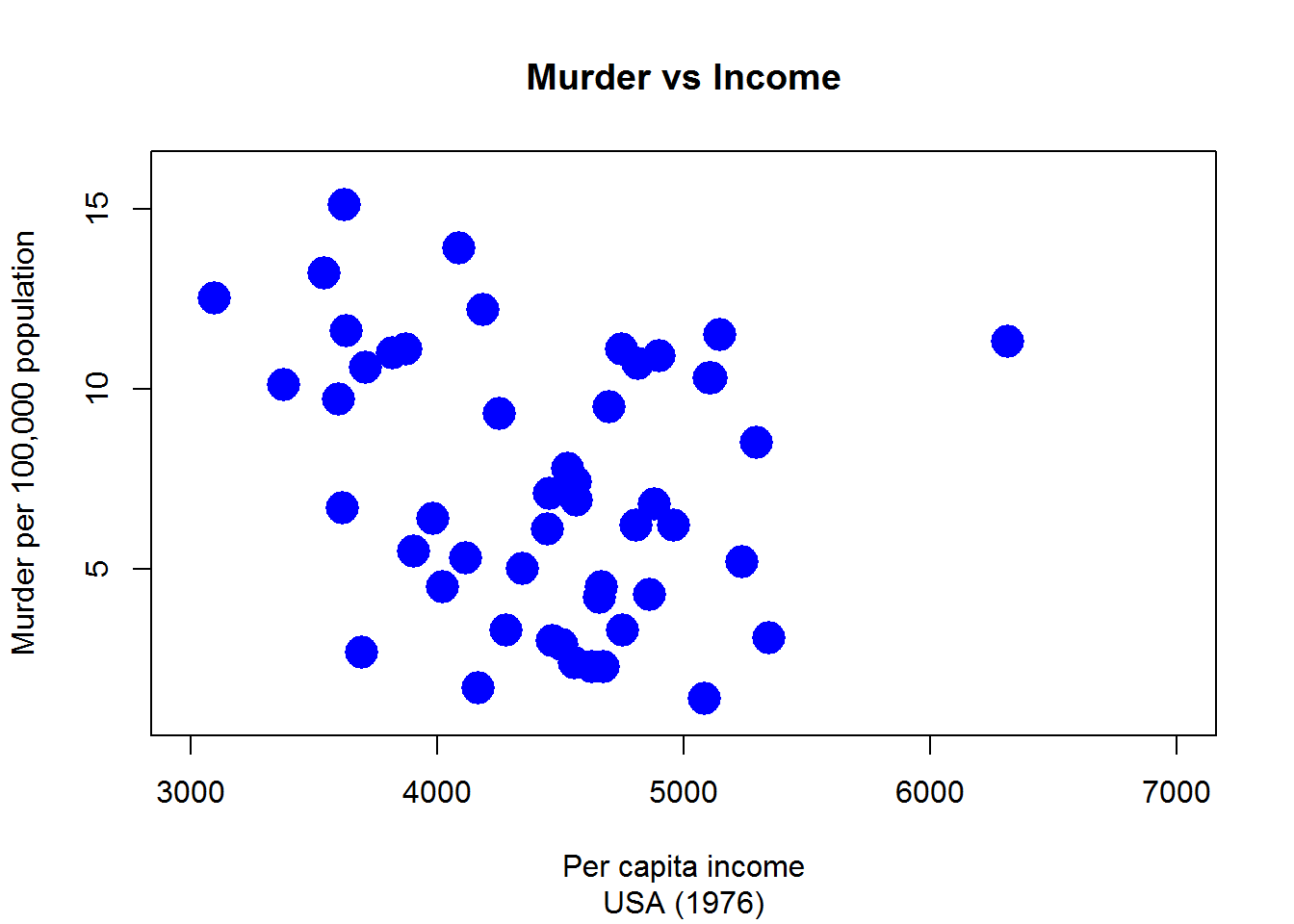The axes can be set on a logarithmic scale with the log parameter. In particular, log = "x" and log = "y" set the x and y axes on a logarithmic base respectively. Use log = "xy" if both axes are to be logarithmic. In the Figure above the y-axis of the graph on the left is in natural scale, whereas the plot on the right shows a y-axis on a logarithmic scale. The graph on the right is generated by: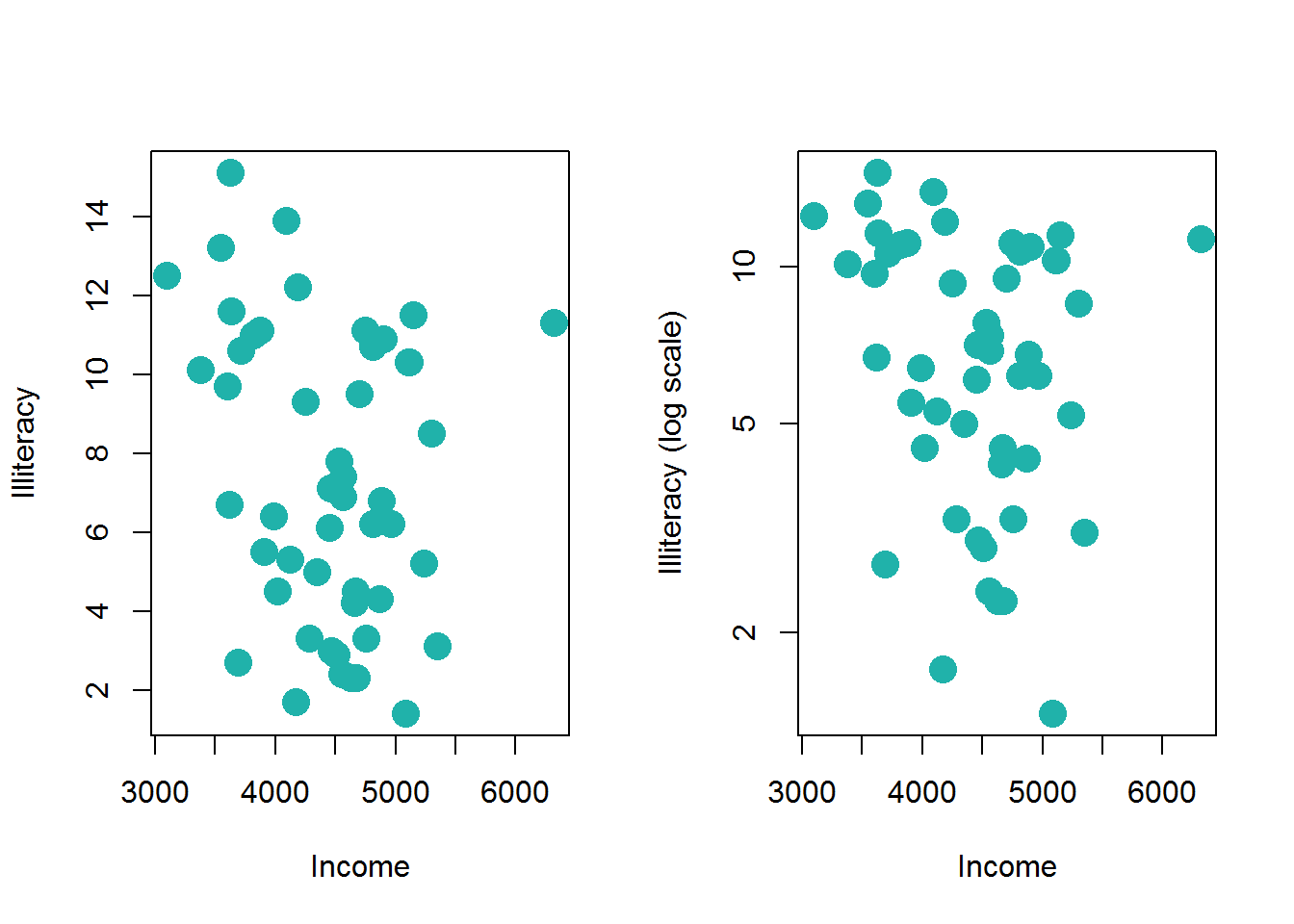## Low-level Functions

So far the main parameters of the plot() functions have been dealt with. However, there are low-level functions which add information to top-level functions. Low-level functions only exist in association with a top-level function, which, in this case, is the plot() function. In the following paragraph it will be shown how some low-level functions can be used to improve the appearance of the graph and the information contained in the scatter plot.

### Text

A text can be inserted inside a scatter plot by specifying the coordinates. text() is a low-level function which introduces some text inside a graph. The input parameters of the text() function are:

• a vector with x coordinates $$(x_1, \dots ,x_n)$$,
• a vector with y coordinates $$(y_1, \dots ,y_n)$$,
• a vector with the text to be inserted.

Clearly, the three above-mentioned vectors need to have the same length. Therefore, the text in the $$i$$-th position will be inserted in the Cartesian coordinate system in $$(xi, yi)$$.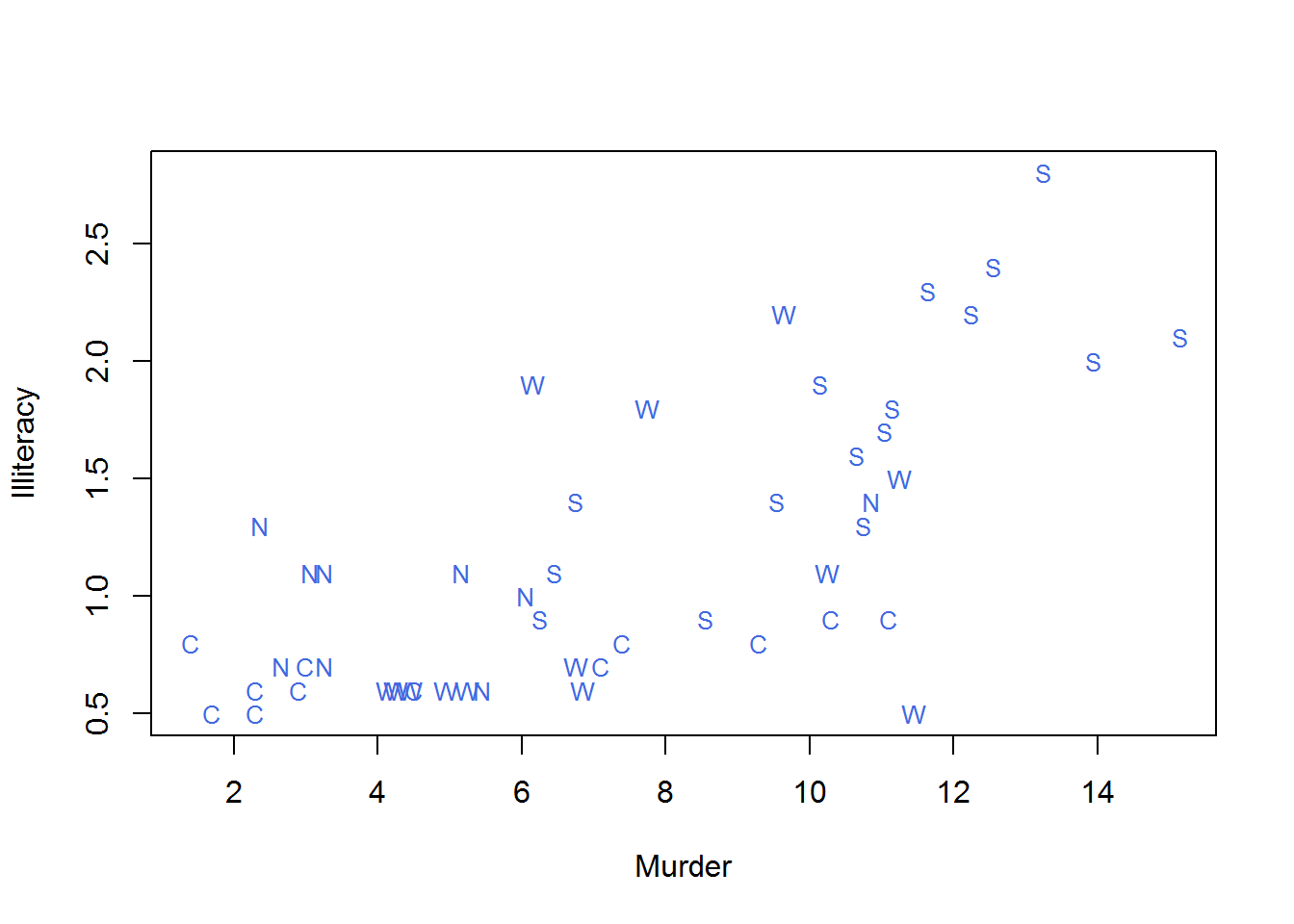If the instructions in code above are analysed, it becomes clear that the type = "n" parameter creates an empty plot, but later on the text() function adds the text of the states.region.abb variable according to the coordinates provided by the Murder and Illiteracy variables. Some parameters of the text() function have been used to customise the output text. The ylim parameter defines the colour, whereas the cex parameter manages the size of the text. The text() function inserts generic text inside the plot. The coordinates defined in the text() function might have no links with those defined in the plot() function. An example is provided below.

In the Code below one of the arguments of the text() function is the adj parameter. The attribute of the adj parameter is a vector with two elements comprised between zero and one. These values indicate the horizontal and vertical alignment of the text (specified by labels) with its x and y coordinates. Some examples of alignment are reported below:

• adj = c(0,0) indicates an alignment on the bottom left.
• adj = c(0.5,0.5) indicates a central position compared with the x and y axes.
• adj = c(1,1) indicates an alignment on the top right.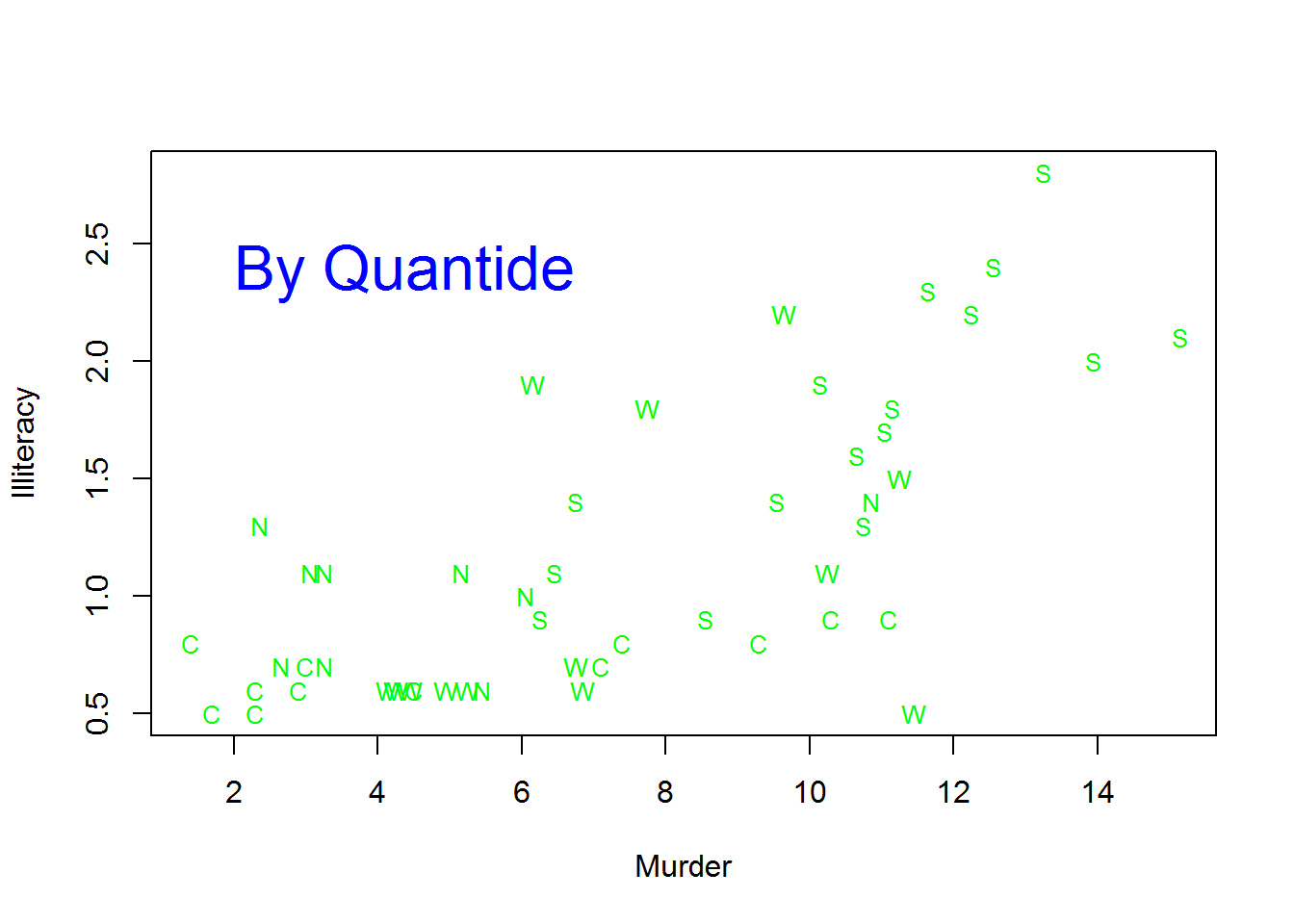### Points

The points() function enables a better control over the symbols used in the scatter plot. The scatter plot below can be created with the instructions of one of codes below.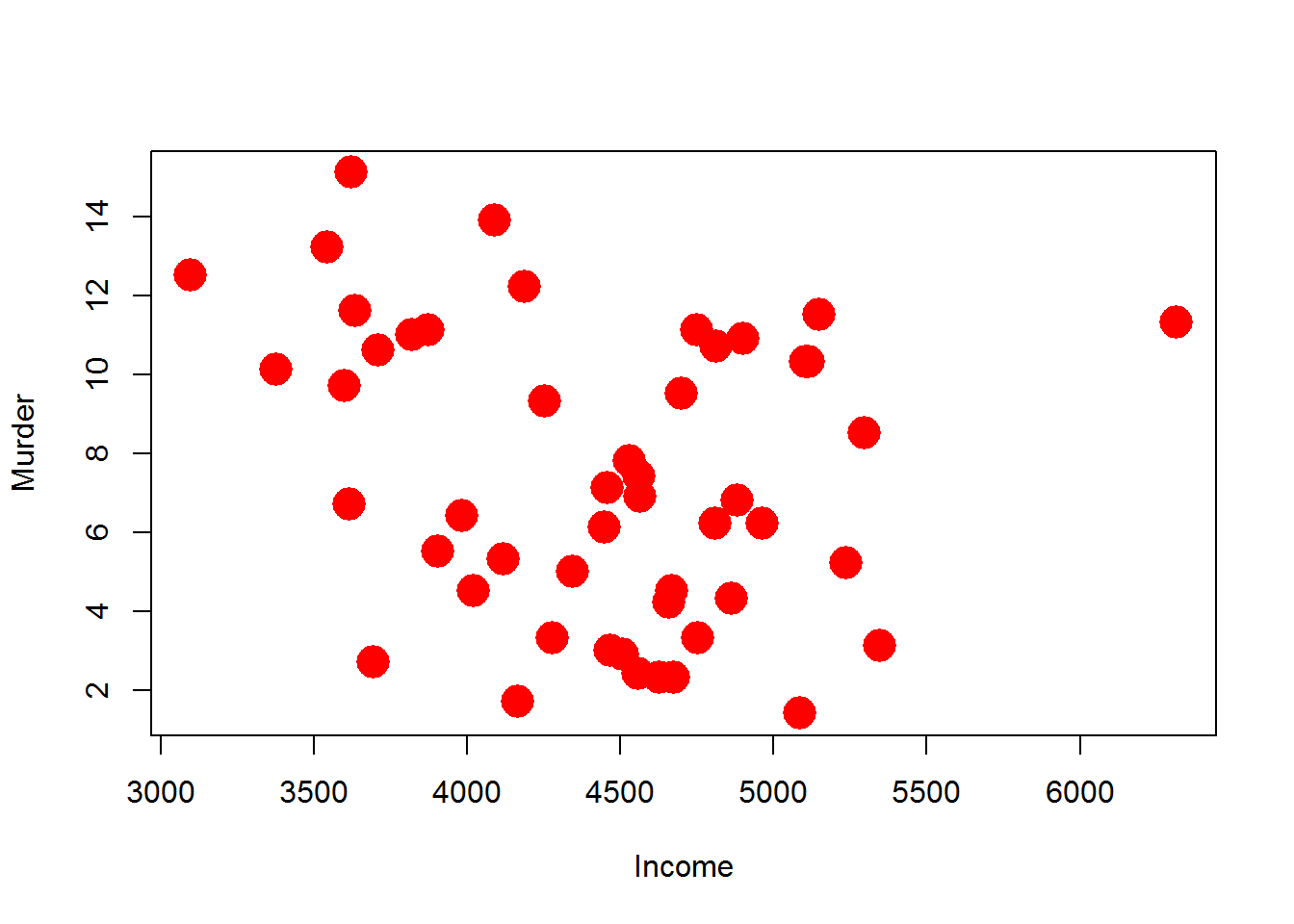The difference between the methods lies in the points not being immediately created by the text() function but being added later thanks to the points() low-level function.

The points() function enables the management of the symbols in terms of a third variable. The instructions contained in Code below provide an example of that kind of use.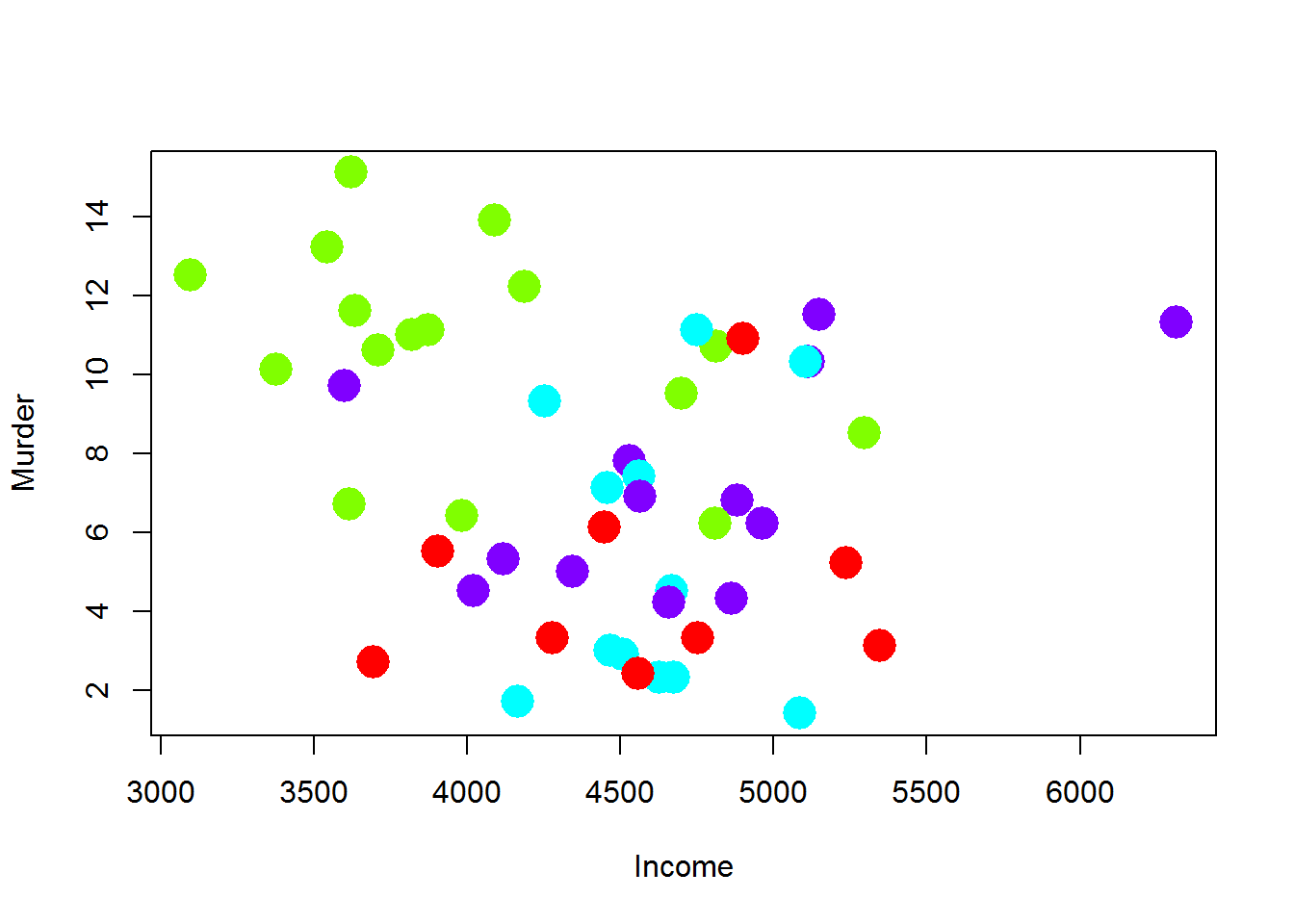The myCol variable is defined as a character vector with the same length as states.region.abb. The elements of myCol are the hexadecimal values of the four colours created by the rainbow(4) function. In the points() function, the myCol vector is used to define the colour of each point in terms of the chosen variable.

The cex parameter of the points() function introduces the information coming from another variable into the plot. As a matter of fact, it is possible to change not only the colour in relation to a variable, but also the dimensions of the points.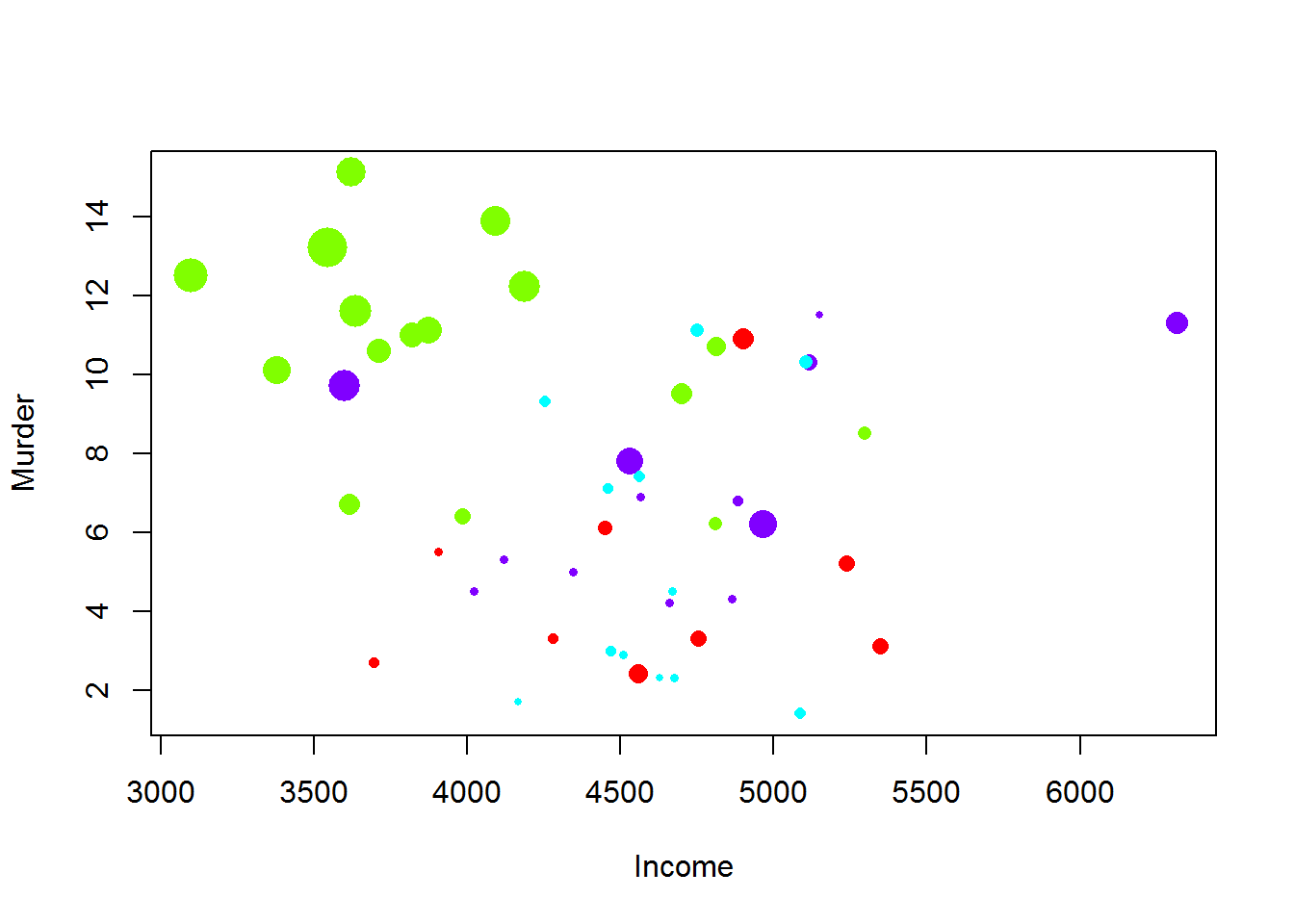### Lines

In a generic plot, lines can be added with the abline() and lines() functions. With sufficient parameters the abline() function draws a straight line in the graph. Horizontal and vertical straight lines are drawn with the abline() function by specifying the h and v parameters respectively. For example, h = 4 draws on the Cartesian coordinate system a straight horizontal line with the equation $$y = 4$$. On the other hand, v = 7 draws on the Cartesian coordinate system a straight vertical line with the equation $$x = 7$$. Oblique lines are created by the abline() function with the a and b parameters which respectively indicate the slope and the intercept of the desired line. For example, if a = 2 and b = 5 the straight line on the Cartesian coordinate system will have the equation $$y = 2x + 5$$.

The reg parameter of the abline() function accepts any regression object with a coefficients method and uses the coefficients to draw the line.

The lines() function joins a set of x and y coordinates using lines. This function is essentially identical to the points() function but its default value is type = "l", instead of type = "p". The lty, lwd and col parameters determine the line type, width and colour in both the abline() and lines() functions. Figure below shows six types of lines created by the lty parameter.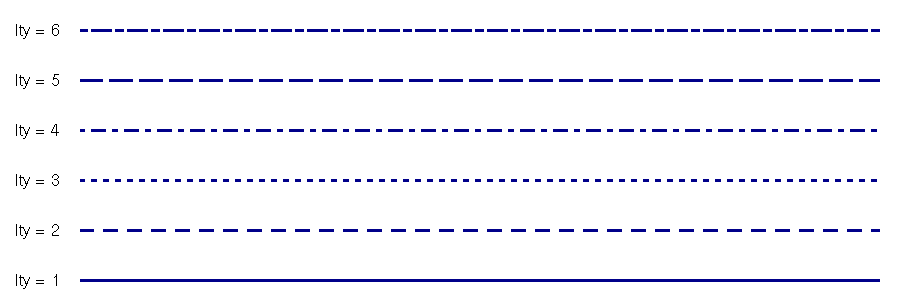The following Code contains an example of the use of the lines() and ablines() functions.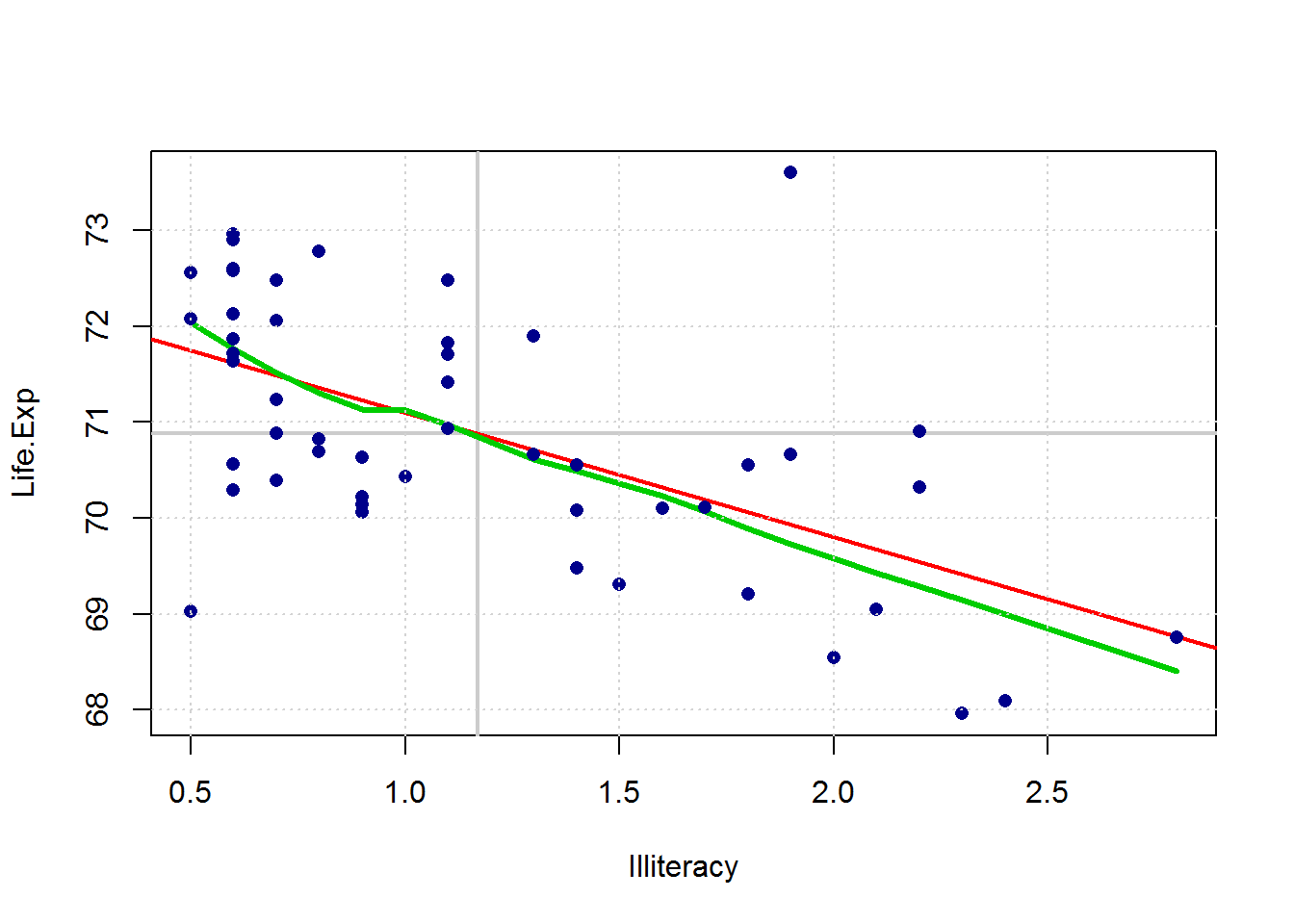In the example, the abline function is firstly used to express the h and v parameters. In the second use of the function the lsfit argument has been specified. The lines() function has drawn the local regression line. The abline() function does not accept an object produced by the lowess() function. This happens because a coefficients method does not exist for this model. By default, the grid() function draws a grid which aligns with the tick marks on the axes. A smaller or larger grid can be obtained specifying the nx and ny parameters which determine the number of vertical and horizontal lines respectively. The grid can be better controlled with the explicit use of abline(). The points() function has been used at the end of the code to prevent points from being hidden by a line drawn in the plot.

### Legend

When symbols with different colours, dimensions and shapes are used in a plot, a legend is needed. In R the legend() function inserts a legend which can be highly customized. The input parameters of the legend() function are x and y. They determine the coordinates where the box with the legend will be inserted. More specifically, the coordinates define the position of the top-left corner of the box. The location of the legend is usually specified by the x parameter only, using the following values:

The inset parameter, as a fraction of the plot region, defines the distance of the legend from the plot margins. A single value refers to the margin of the x-axis. Two values, on the other hand, are referred to the margins of the x and y axes. The legend parameter defines the legend text. The ncol parameter sets the number of columns of the legend; if it is not specified the legend will have only one column. The width and the line type of the legend box can be set using the box.lwd and box.lty parameters. Thebty = "n" parameter eliminates the margins of the legend. The title parameter inserts a title for the legend. As it can be seen, there are no limits as to how many legends can be inserted in a plot.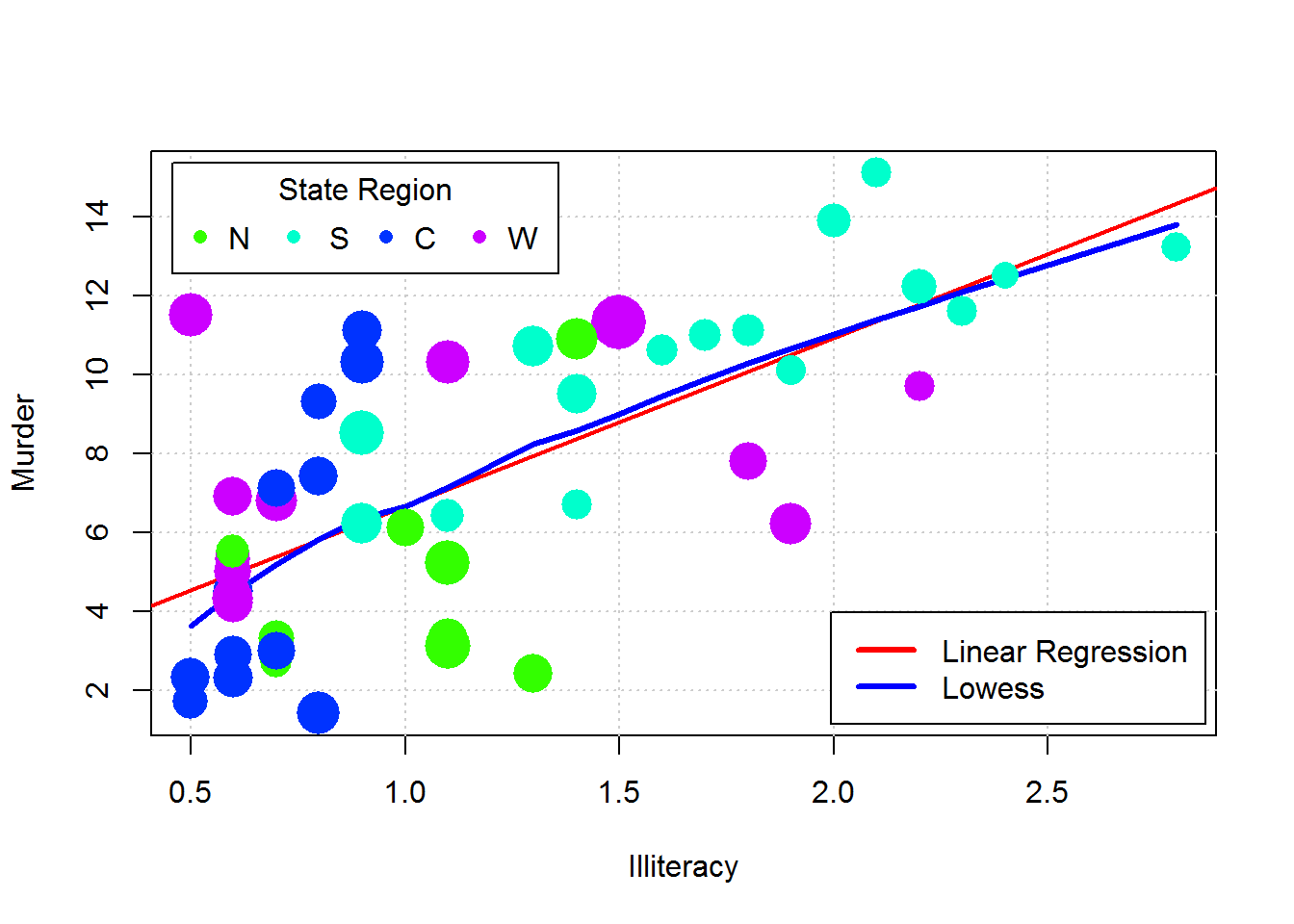### Titles

title() is a low-level function which inserts the title in a plot. There are four different positions for a title in a graph:

1. top-centre
2. bottom-centre
3. as label for the x-axis
4. as label for the y-axis

If the outer parameter is set on TRUE, the title will be placed in the outer margin of the plot. The cex parameter controls the size of the title. The function is usually used to define main titles, whereas axes labels are managed with the xlab and ylab parameters of the plot() function or the label parameter of the axis() function. The “\n” symbol inside the string of the main title splits the title over two lines.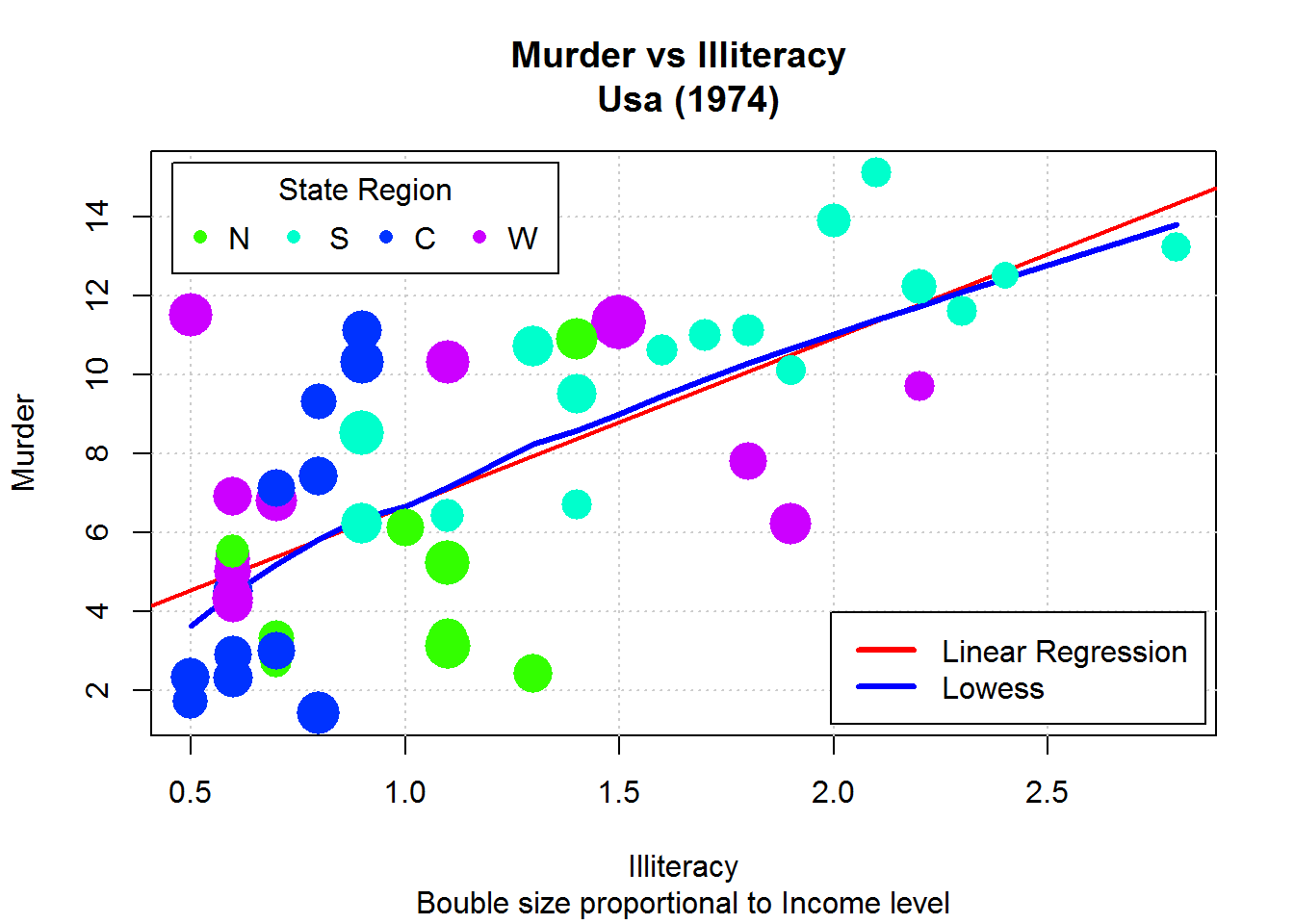### Polygons

The polygon() function is used to draw a polygon in a plot. The basic arguments of the polygon() function are the x and y vectors which contain the coordinates of the vertices of the polygon. Therefore, the x and y arguments are numerical vectors with the same length. With this function the polygon is created by uniting the coordinates given in progression and is closed by joining the last point to the first.

In Code above x-values are defined from a vector with a length of $$n/2$$ in ascending order and linked to the vector itself in a descending order. In this way, the vector will be $$n$$-long, as the ordinate vector. This method will ensure the correct closure of the polygon.

The polygon() function can be useful to draw a confidence interval for the regression line.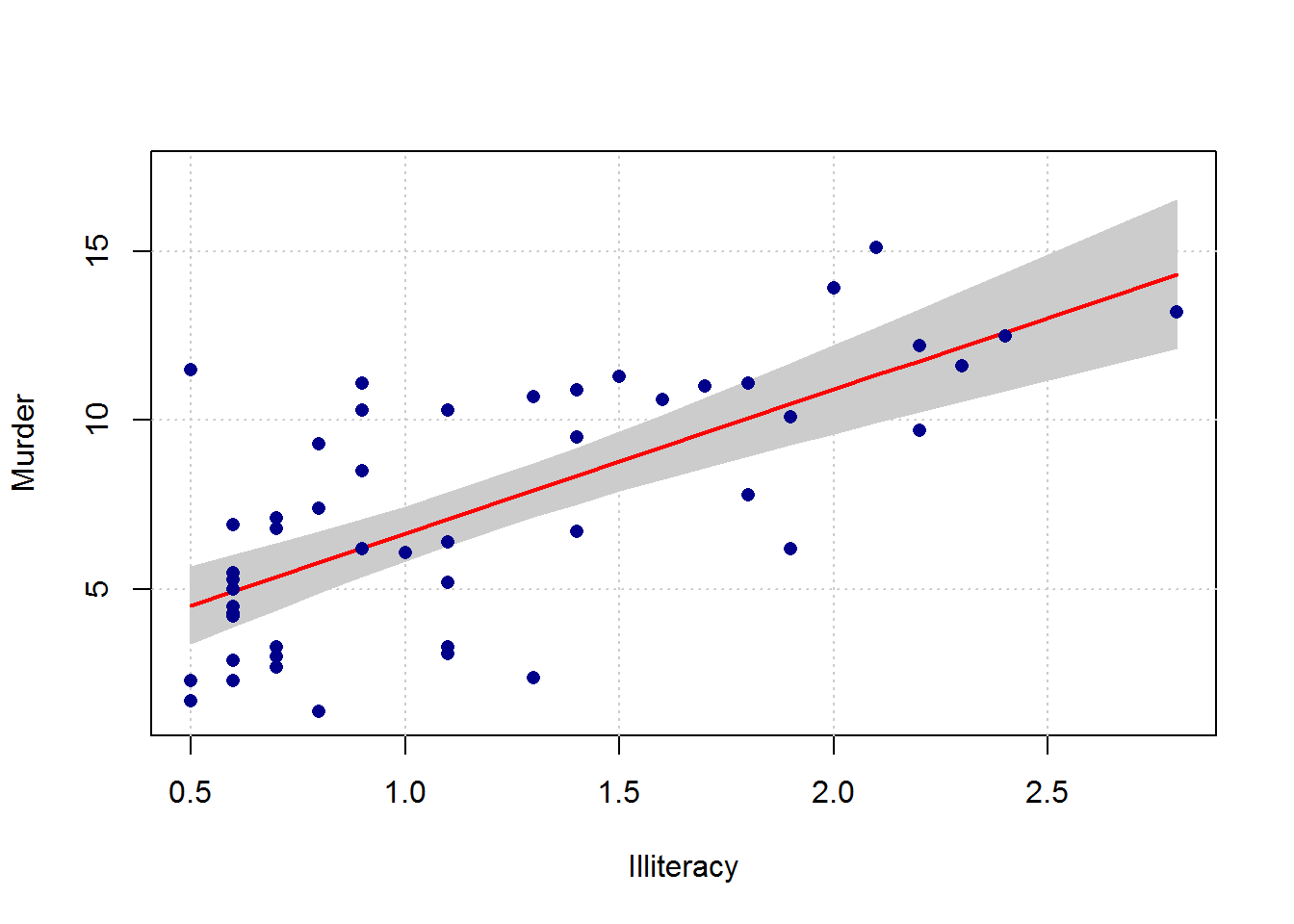A simple linear model has been estimated in Code above. The predict() function creates the matrix which contains the upper and lower limit of the confidence interval. In the predict() function the newdata parameter has been defined to order the values of confidence limits according to the values of the Illiteracy regressor.

Besides properly defined coordinates, the polygon() function also uses the col and border arguments to chose the area and border colours of the polygon. The limits of the y-axis have been redefined for them to be sufficiently wide to contain the whole confidence interval. Finally, the polygon has been created in the plot before drawing the points to prevent them from being hidden. The lines() function draws the regression line.

### Axes

The axis() function adds one or more axes to the plot. With the axis() function it is possible to specify location, density and labels. There are also numerous other functions. In particular, the side option determines the position of the axis: 1 = below, 2 = left, 3 = above and 4 = right. This parameter is obviously mandatory. An example of the application of the axis() function is provided in Code below. It is also shown how to create the grid in reference to the range defined by the new axes. Beside the side parameter, other fundamental arguments of the axis() function are at and labels. The at parameter defines the new location of the axes labels. The labels arguments indicates the character to be printed in each position. Clearly, the two vectors associated with the at and labels parameters need to have the same length.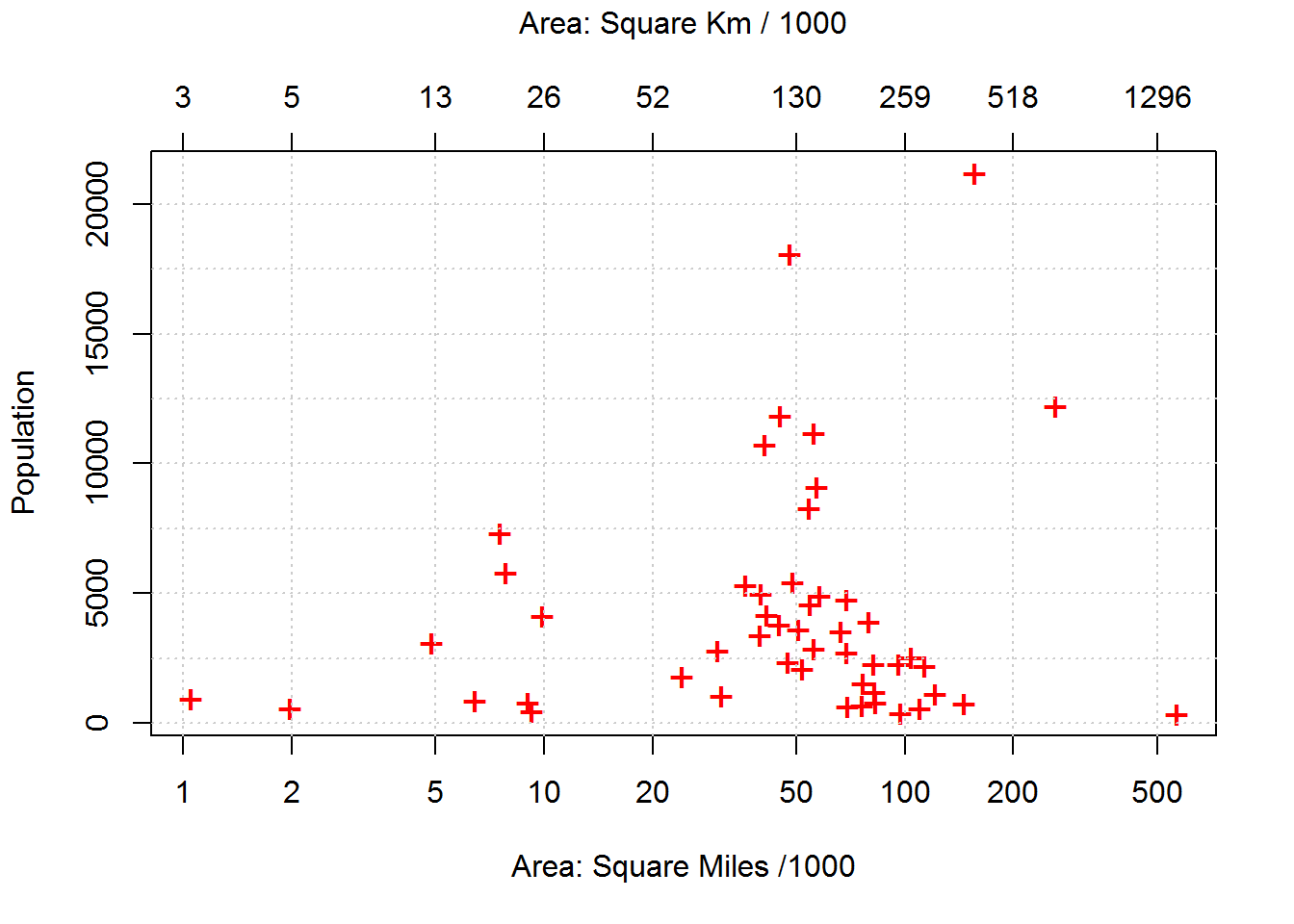The parameters of the axis() function are used to modify the axes default settings, such as order, colour and dimensions. Code below produces the same basic plot as Code above, but different styles have been applied to the axes. In particular, the label orientation has been modified using the las parameter and the colours of labels and axes have been changed with the col and col.axis parameters respectively. The already-discussed lty, cex and lwd parameters do not define the features of lines and points inside the plot, but are used to manage the characteristics of lines and labels created by the axes.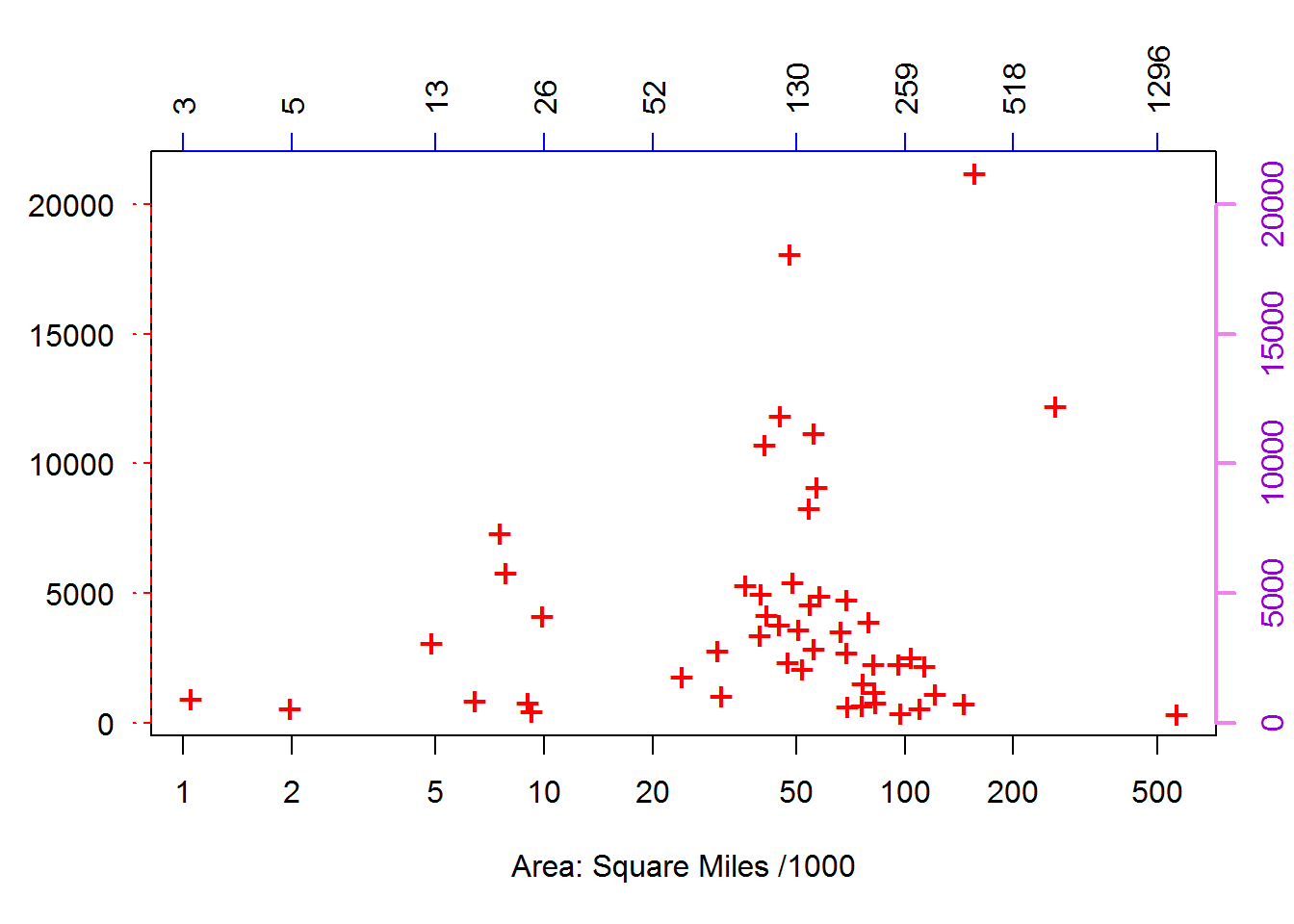## Histograms, Barplot, Boxplot and Three Dimensional Plots

### Histograms

A histogram is a representation of a frequency distribution by means of rectangles whose widths represent class intervals and whose areas are proportional to the corresponding frequencies.

When the freq argument of hist() is set to FALSE probability densities are plotted so that the histogram has a total area of one.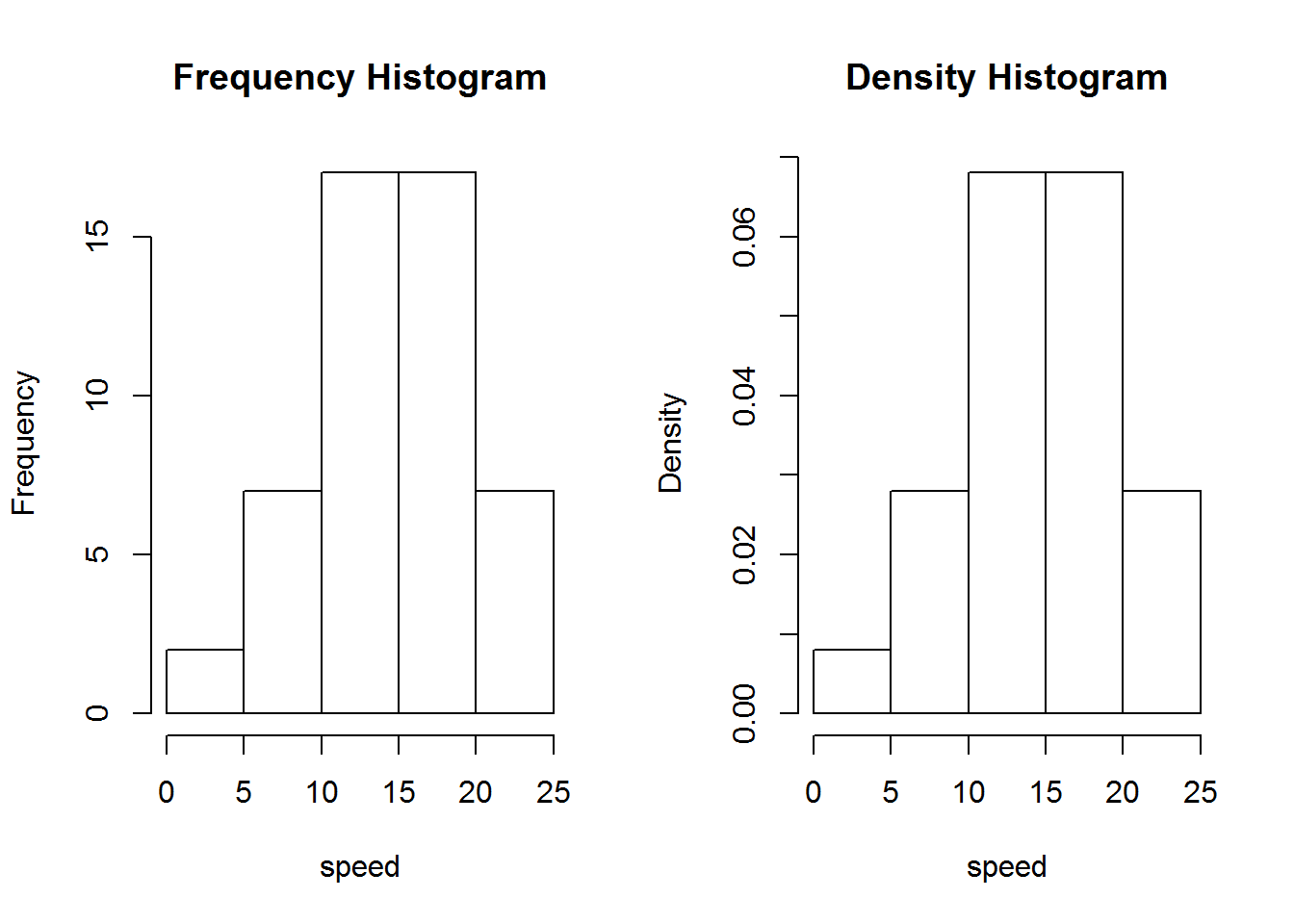The number of breaks/classes is automatically determined but can be defined if required by specifying either the numbers of classes or the break points: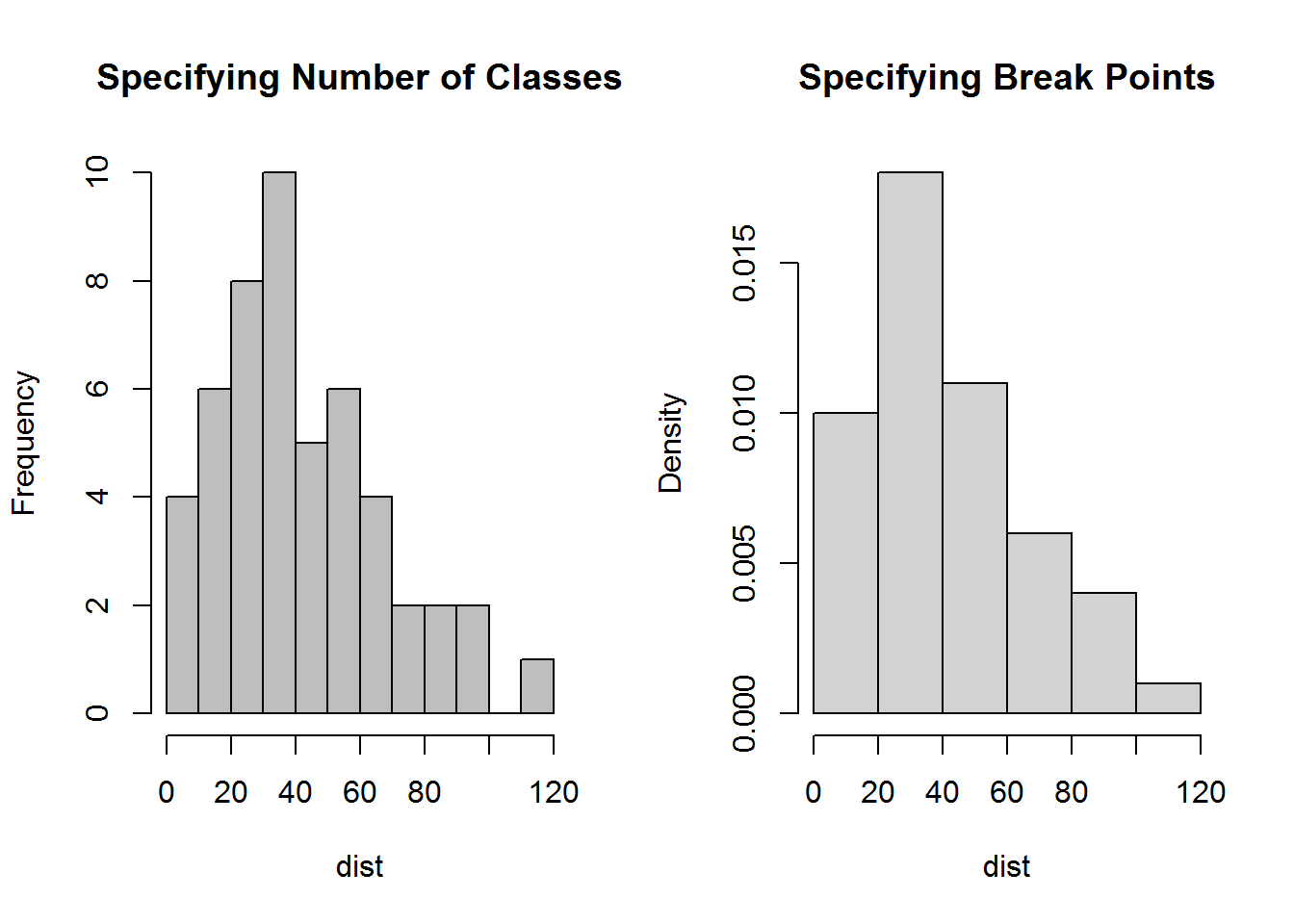### Barplot

A bar plot displays the frequencies (or relative frequencies) for categorical variables. Generally, a grouping function such as table() is applied to data prior to draw barplots.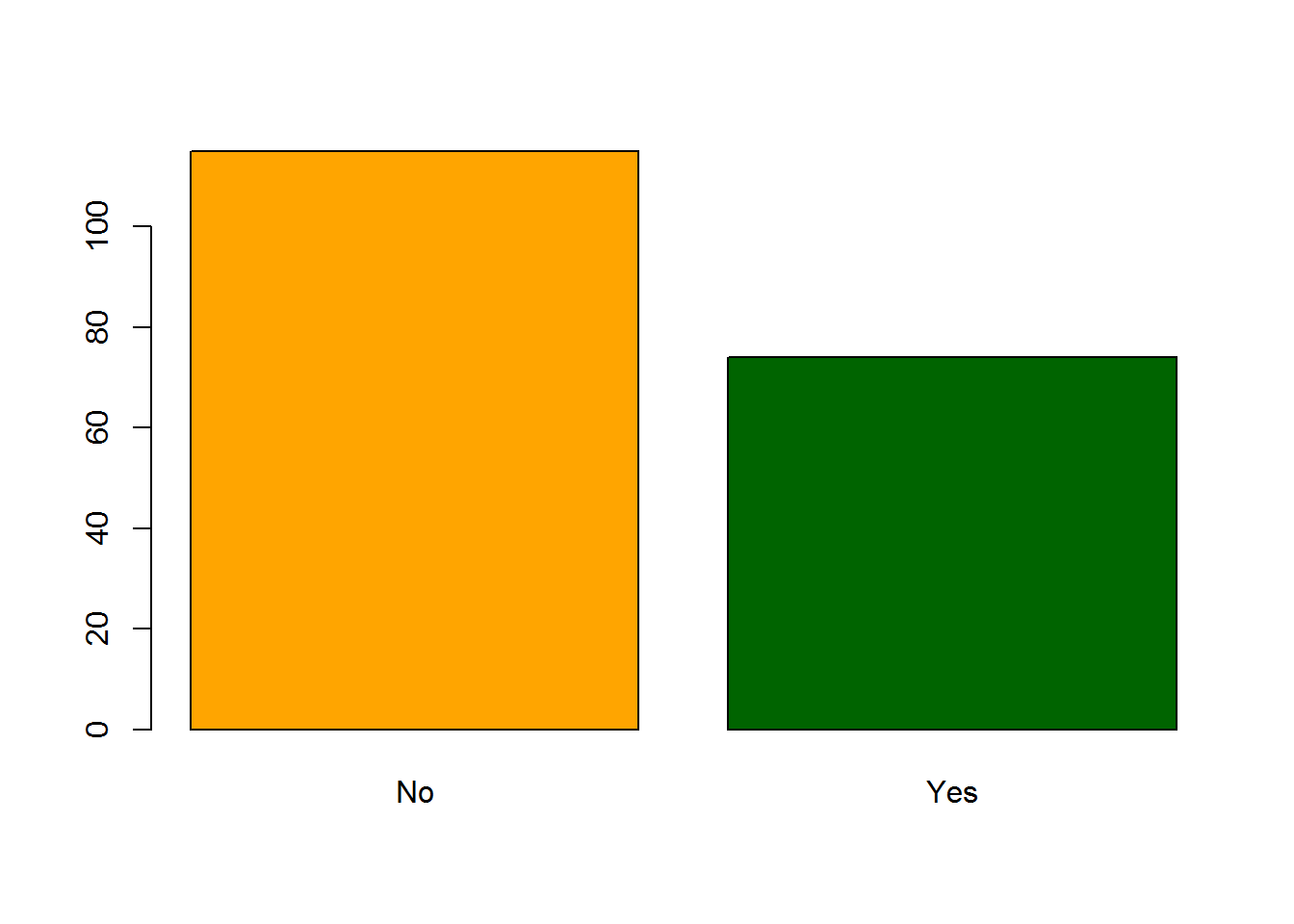When introducing two or more variables, barplots can be constructed in stacked or beside mode. A simple legend may added to the plot by setting to TRUE the legend argument.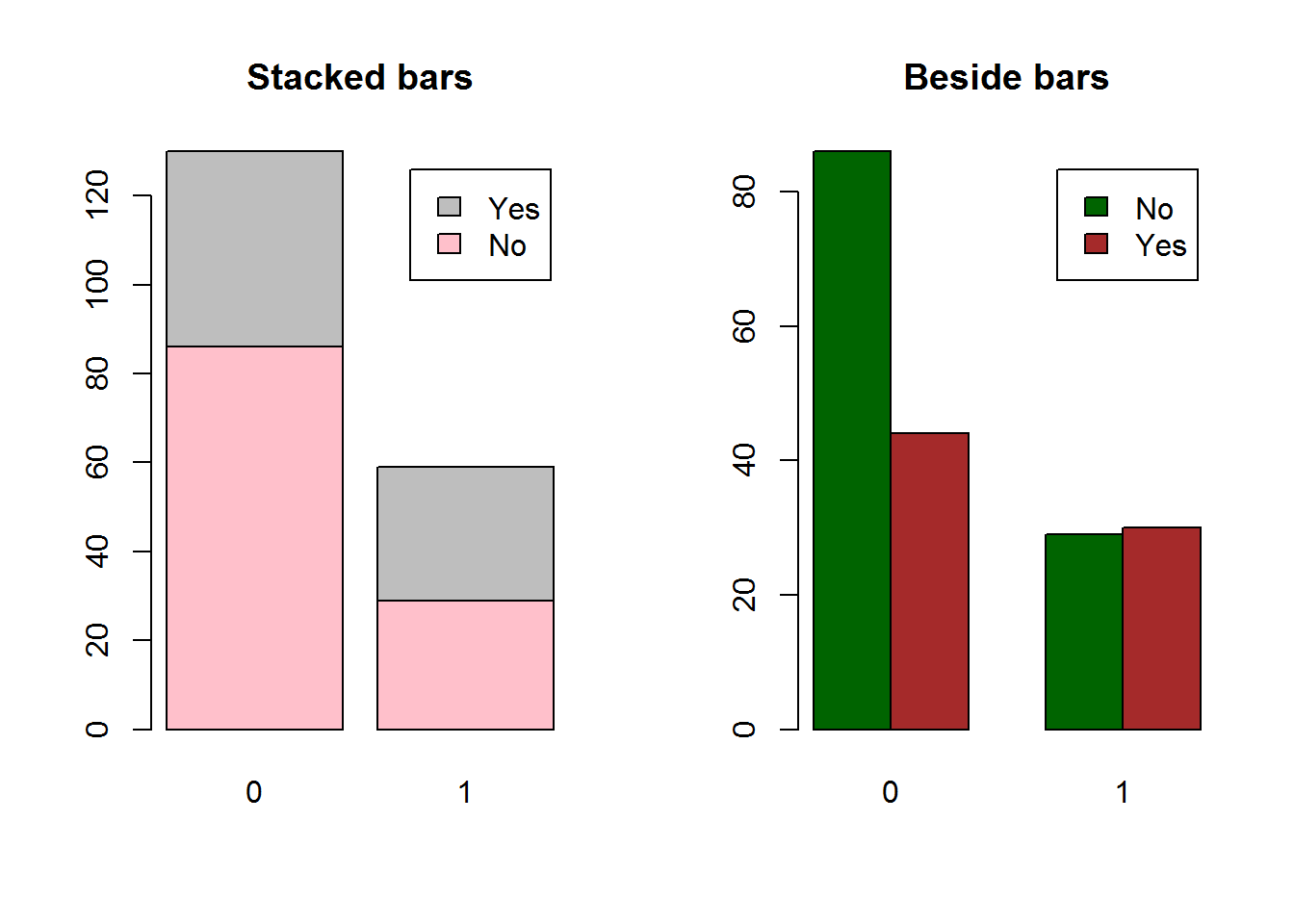### Boxplot

“Box and whiskers” plots, often called boxplots, are a way of summarizing and comparing data distributions.

The “box” in a boxplot shows the median as a line and the first (25th percentile) and third quartile (75th percentile) of the distribution as the lower and upper parts of the box.

The “whiskers” shown above and below the boxes technically represent the largest and smallest observed data that are less than 1.5 box lengths from the end of the box. In practice, these data are about the lowest and highest values one is likely to observe. Data above or below whiskers are shown as open circles “o” or stars.

In comparing the boxplots across groups, a simple summary is to say that the “box” area for one group is higher or lower than that for another group.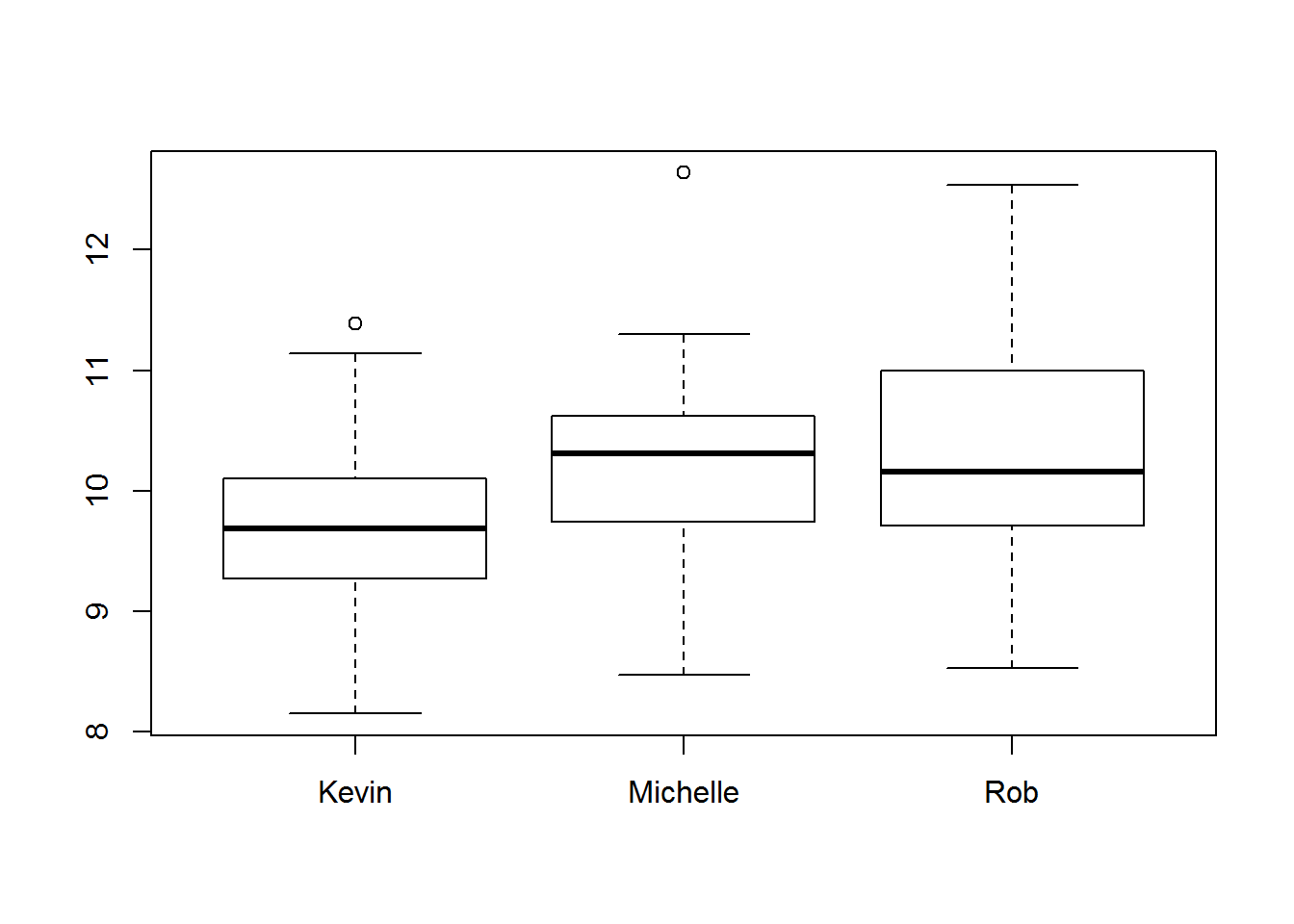Formula method seems to be the only alternative unless reshaping data in wide format before drawing the boxplot.

### Three Dimensional Plots

Three dimensional graphics are quite fashionable and good looking. Nevertheless, more technical two dimensions plots such as trellis graphics may help to understand graphics in better details.

R offers a wide variety of three dimensional graphics. Some not exaustive examples when representing a bivariate normal distribution are: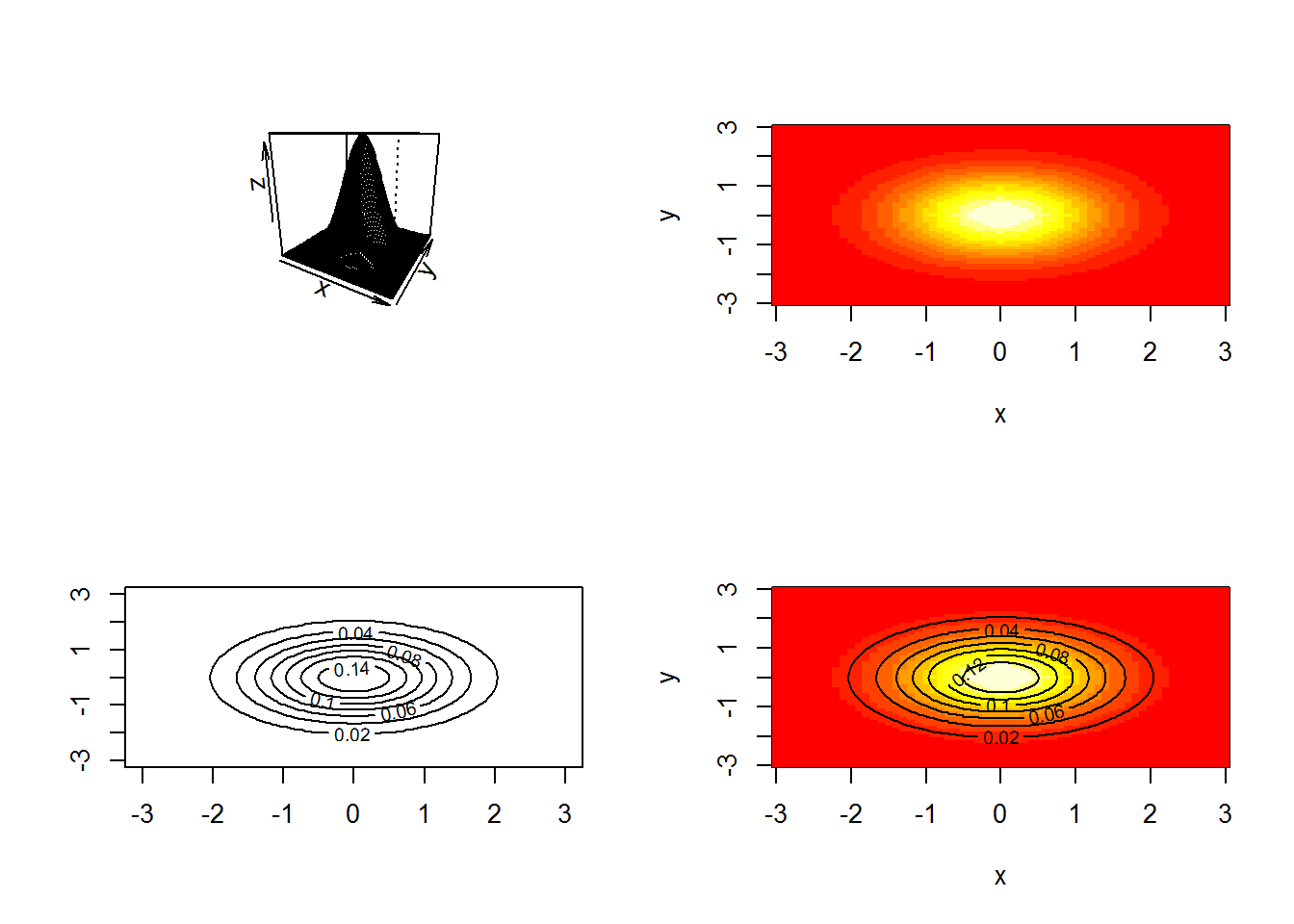By using a different technique from the lattice package. The lattice package will be presented in the next Paragraph.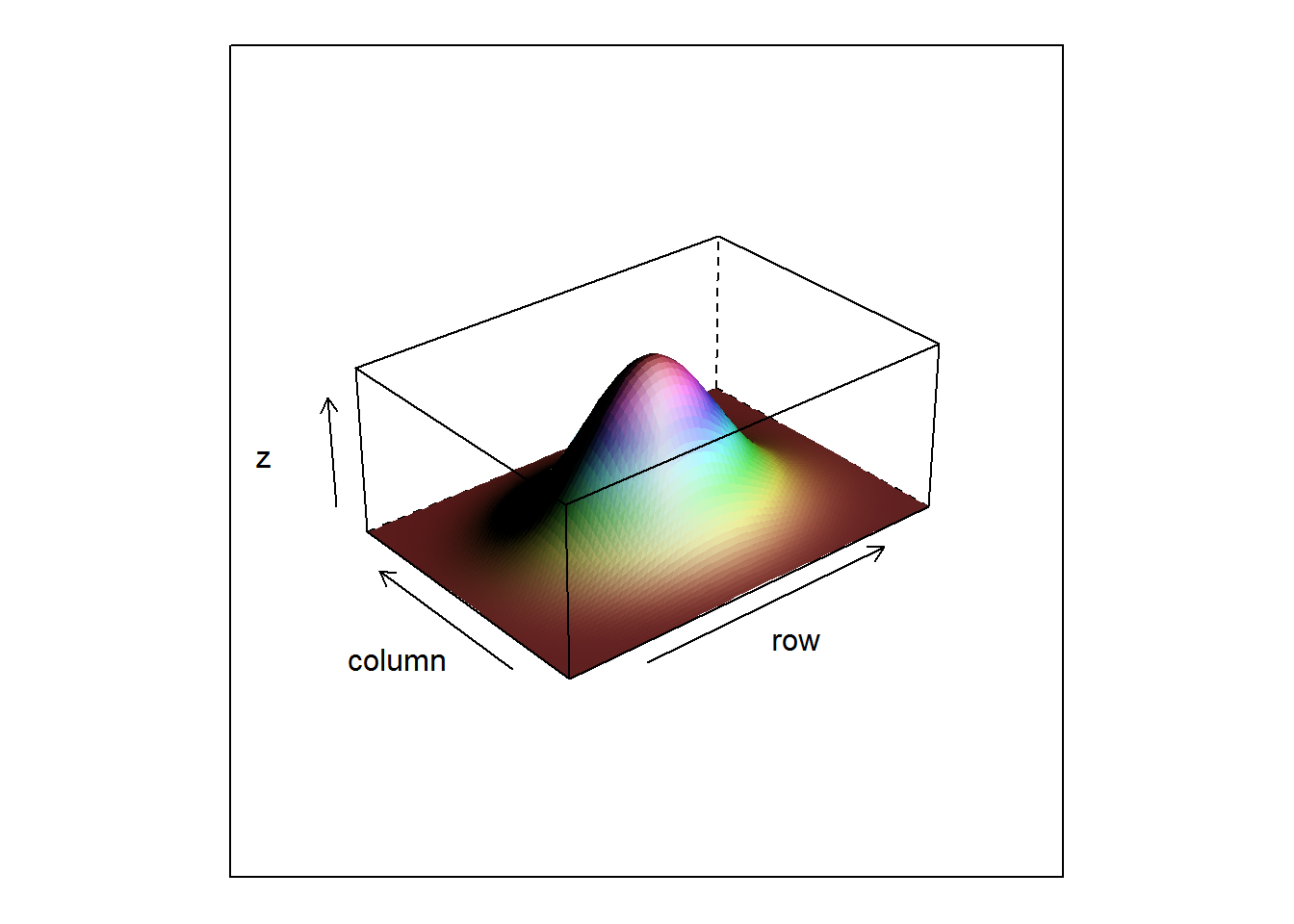## Introduction to Alternative Graphic Systems

### lattice Graphics

lattice is an add-on package that implements Trellis graphics (originally developed for S and S-Plus) in R.

It is a powerful and elegant high-level data visualization system, with an emphasis on multivariate data, that is sufficient for typical graphics needs, and is also flexible enough to handle most nonstandard requirements.

Standard lattice type of graphics include:

A lattice display is usually takes two arguments:

• a formula object;
• a data frame.

Formulas are generally defined as: $$y \sim x | f$$ meaning to plot y versus x in a separate panel as defined by the level of factor f.

Plot customization is made by mean of the panel argument. Panel argument require a function, usually built by combining standard panel functions defined as part of the lattice package.

The istat dataset contains information about weight and height for females and males. The interests is in understanding in which proportion weight is explained by height and how this relatioship differs from females to males.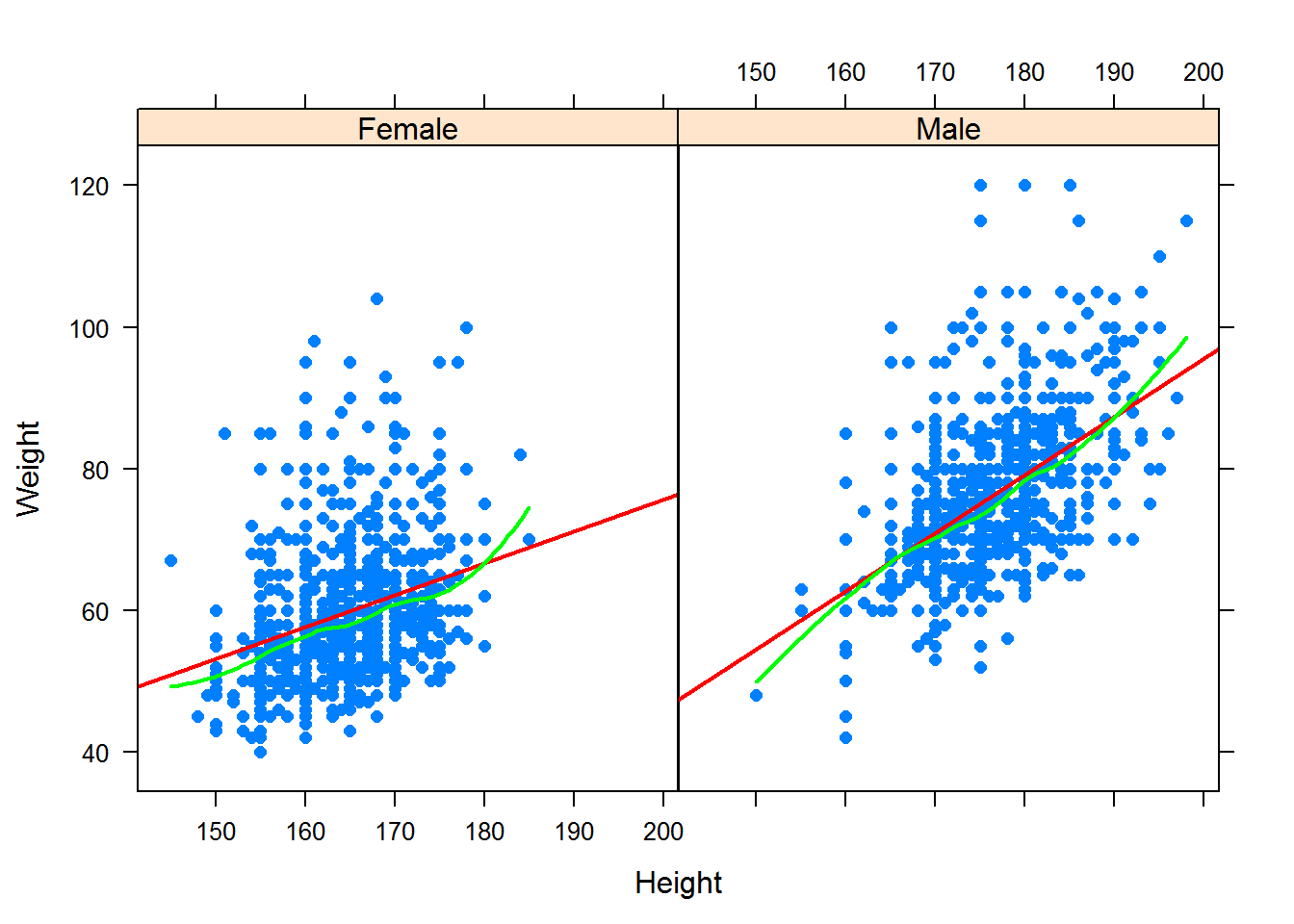### ggplot2 Graphics

ggplot2 is a plotting system, based on the grammar of graphics, which tries to take the good parts of base and lattice graphics and none of the bad parts.

It takes care of many of the fiddly details that make plotting a hassle (like drawing legends) as well as providing a powerful model of graphics that makes it easy to produce complex multi-layered graphics.

A full explanatory introduction to the ggplot2 package is available at: had.co.nz/ggplot2.

The dataset spc contains one-hundred measurements from an industrial process. Data were collected hourly in groups of size equal to four. The engineers wants to produce an xbar control chart: a very common chart used to track a series of sample averages over time.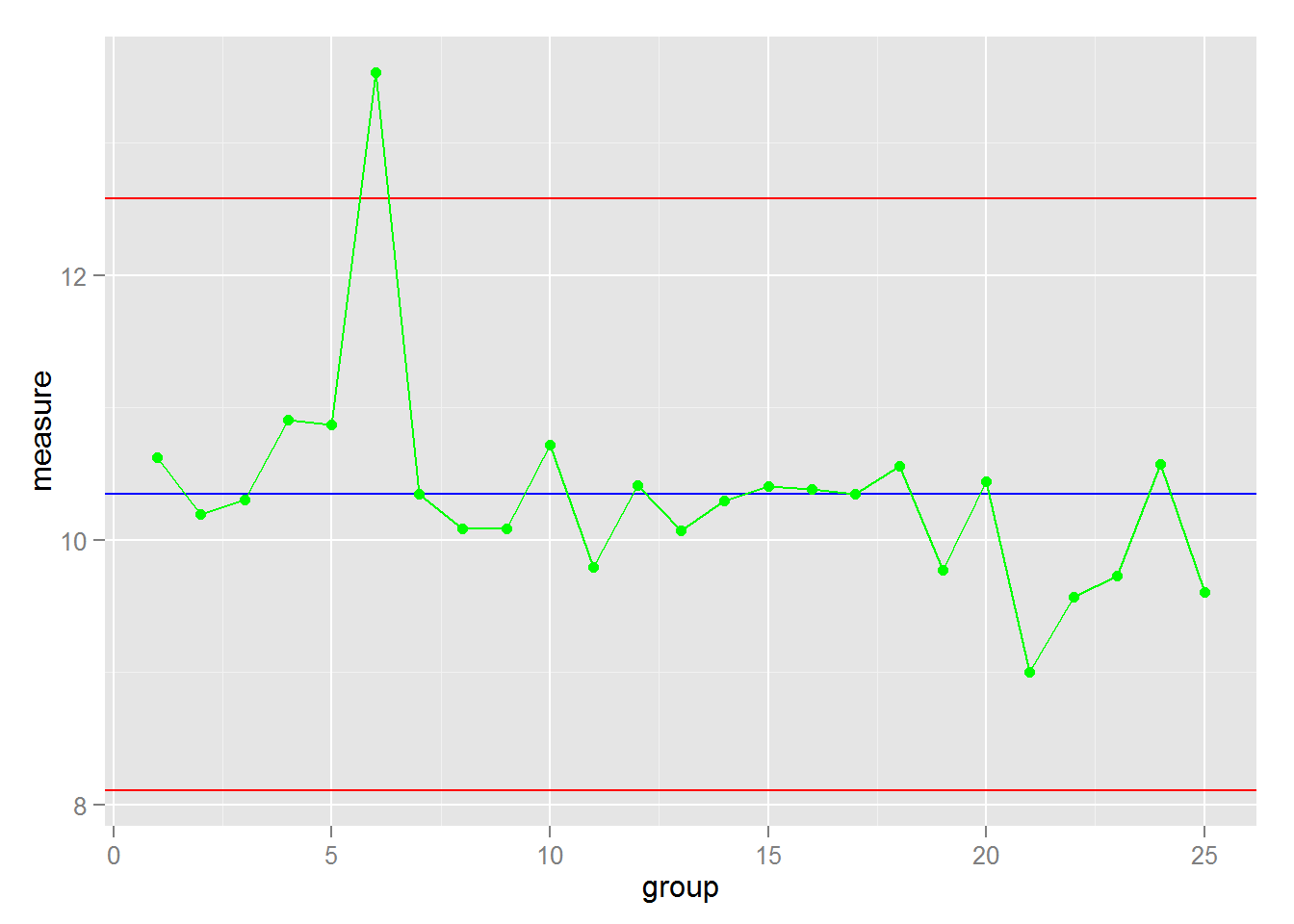## Summary

In this chapter, we explored the graphical potentiality of R. We introduced the graphic environment, differentiating between low and high level plot functions. We drew a scatter plot and learned how to modify points type, size and colour, add titles, points, lines, and legends, modify axes. We explored how histograms and box plots can help us visualise the distribution of continuous variables. We saw how bar plots can be used to gain insight into the distribution of a categorical variable, and how stacked and grouped bar charts can help us understand how groups differ on a categorical outcome. We took a look to alternatives graphic systems lattice and ggplot2. In the next chapter, you’ll write your own function with R!